# Blog

## How To Draw The MandelBrot Set In Google Sheets, Using Only Formulas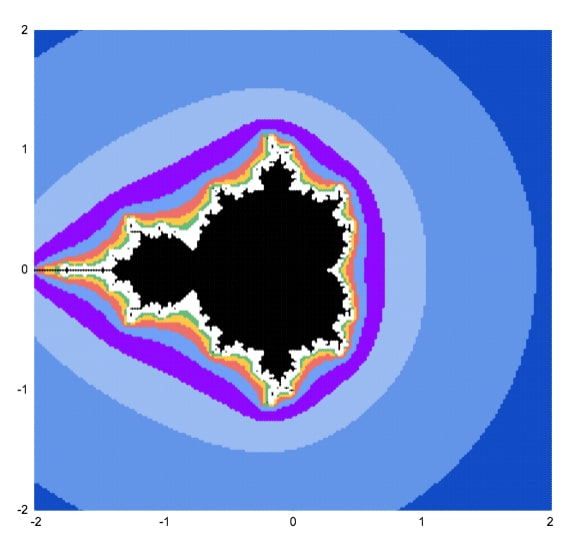This article will walk you through how to draw the MandelBrot set, using only formulas and the built-in chart tool.

## What Is The MandelBrot Set?

The Mandelbrot set is group of special numbers with some astonishing properties.

You’ve almost certainly seen an image of the Mandelbrot set before.

It’s one of the most famous mathematical concepts, known outside of mathematical circles even. It’s named after the French mathematician, Benoit Mandelbrot, who was the father of fractal geometry.

The Mandelbrot set is fantastically beautiful. It’s an exquisite work of art, generated by a simple equation.

More formally, the Mandelbrot set is the set of complex numbers c, for which the equation z² + c does not diverge when iterated from z = 0.

Gosh, what does that mean?

Well, it’s easier if you look at the picture at the top of this article. The black area represents points that do not run away to infinity when you keep applying the equation z² + c.

Consider c = -1.

It repeats -1, 0, -1, 0, -1… forever, so it never escapes. It’s bounded so it belongs in the Mandelbrot set.

Now consider c = 1.

Starting with z = 0, the first iteration is 1.

The second iteration is 1² + 1 = 2.

The third iteration is 2² + 1 = 5.

The fourth iteration is 5² + 1 = 26.

And so on 26, 677, 458330, 210066388901, … It blows up!

It diverges to infinity, so it is not in the Mandelbrot set.

Before we can draw the Mandelbrot set, we need to think about complex numbers.

### Imaginary And Complex Numbers

It’s impossible to draw the Mandelbrot set without a basic understanding of complex numbers.

If you’re new to complex numbers, have a read of this primer article first: Complex Numbers in Google Sheets

It explains what complex numbers are and how you use them in Google Sheets.

To recap, complex numbers are numbers in the form

`a + bi`

where i is the square root of -1.

We can plot them as coordinates on a 2-dimensional plane, where the real coefficient “a” is the x-axis and the imaginary coefficient “b” is on the y-axis.

### Use A Scatter Plot To Draw The Mandelbrot Set

Taking each point on this 2-d plane in turn, we test it to see if it belongs to the Mandelbrot set. If it does belong, it gets one color. If it doesn’t belong, it gets a different color.

This scatter plot chart of colored points is an approximate view of the Mandelbrot set.

As we increase the number of points plotted and the number of iterations we get successively more detailed views of the Mandelbrot set. A point that may still be < 2 on iteration 3 may be clearly outside that boundary by iteration 5, 7 or 10.You can see the outline resembles the Mandelbrot set much more clearly at higher iterations.

## How To Draw The MandelBrot Set In Google Sheets, Using Only Formulas

Here’s a simple approximation of the Mandelbrot set drawn in Google Sheets: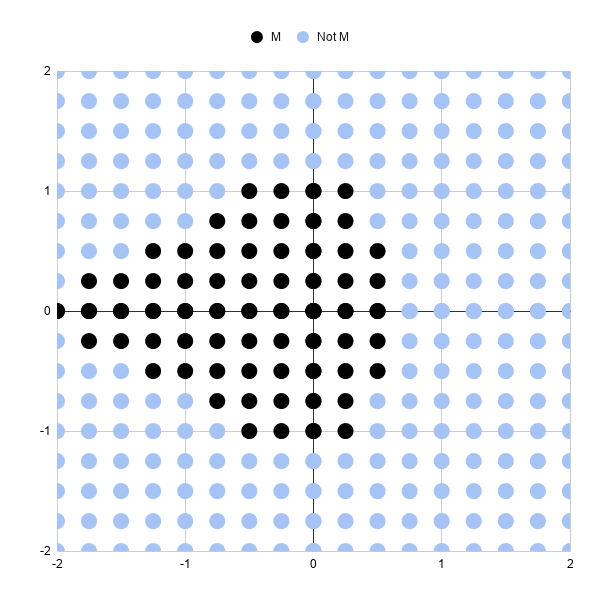Let’s run through the steps to create it.

It’s a scatter plot with 289 points (17 by 17 points).

Each point is colored to show whether it’s in the Mandlebrot set or not, after 3 iterations.

Black points represent complex numbers, which are just coordinates (a,b) remember, whose sizes are still less than or equal to 2 after 3 iterations. So we’re including them in our Mandelbrot set.

Light blue points represent complex numbers whose sizes have grown larger than 2 and are not in our Mandelbrot set. In other words, they’re diverging.

Three iterations is not very many, which is why this chart is a very crude approximation of the Mandelbrot set. Some of these black points will eventually diverge on higher iterations.

And obviously we need more points so we can fill in the gaps between the points and test those complex numbers.

But it’s a start.

### Generating The Complex Number Coordinates

In column A, we need the sequence going from 0 to 2 and repeating { 0, 0.25, 0.5, 0.75, 1, 1.25, 1.5, 1.75, 2, 0, 0.25, 0.5, …}

In column B, we need the sequence 0, then 0.25, then 0.5 repeating 9 times each until we reach 2 { 0, 0, 0, 0, 0, 0, 0, 0, 0, 0.25, 0.25, 0.25, …}

This gives us the combinations of x and y coordinates for our complex numbers c that we’ll test to see if they lie in the set or not.

It’s easier to see in the actual Sheet: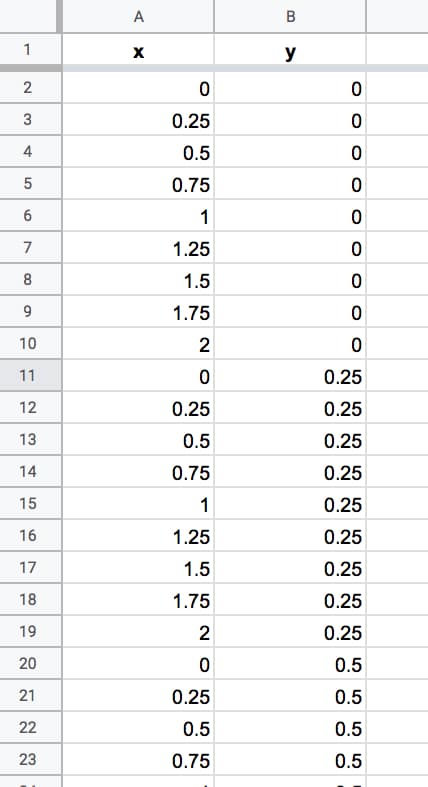We keep going until we reach number 2 in column B. We have 324 rows of data. There are some repeated rows (like 0,0) but that doesn’t matter for now.

Columns A and B now contain the coordinates for our grid of complex numbers. Now we need to test each one to see if it belongs in the Mandelbrot set.

### Formulas For The Mandelbrot Algorithm

We create the algorithm steps in columns C through J.

In cell C2, we create the complex number by combining the real and imaginary coefficients:

`=COMPLEX(A2,B2)`

Cell D2 contains the first iteration of the algorithm with z = 0, so the result is equal to C, the complex number in cell C2, hence:

`=C2`

The second iteration is in cell E2. The equation this time is z² + c, where z is the value in cell D2 and C is the value from C2:

`=IMSUM(IMPOWER(D2,2),\$C2)`

It’s the same z² + c in F2, where z in this case is from E2 (the previous iteration):

`=IMSUM(IMPOWER(E2,2),\$C2)`

Notice the “\$” sign in front of the C in both formulas in columns E and F. This is to lock our formula back to the C value for our Mandelbrot equation.

In cell G2:

`=IMABS(D2)`

Drag this formula across cells H2 and I2 also.

In cell J2:

`=IFERROR(IF(I2<=2,1,2),2)`

Then leave a couple of blank columns, before putting the chart data in columns M, N and O, with the following formulas representing the x values and the two y series with different colors:

`=A2`

`=IF(J2=1,B2,"")`

`=IF(J2=2,B2,"")`

Thus, our dataset now looks like this (click to enlarge):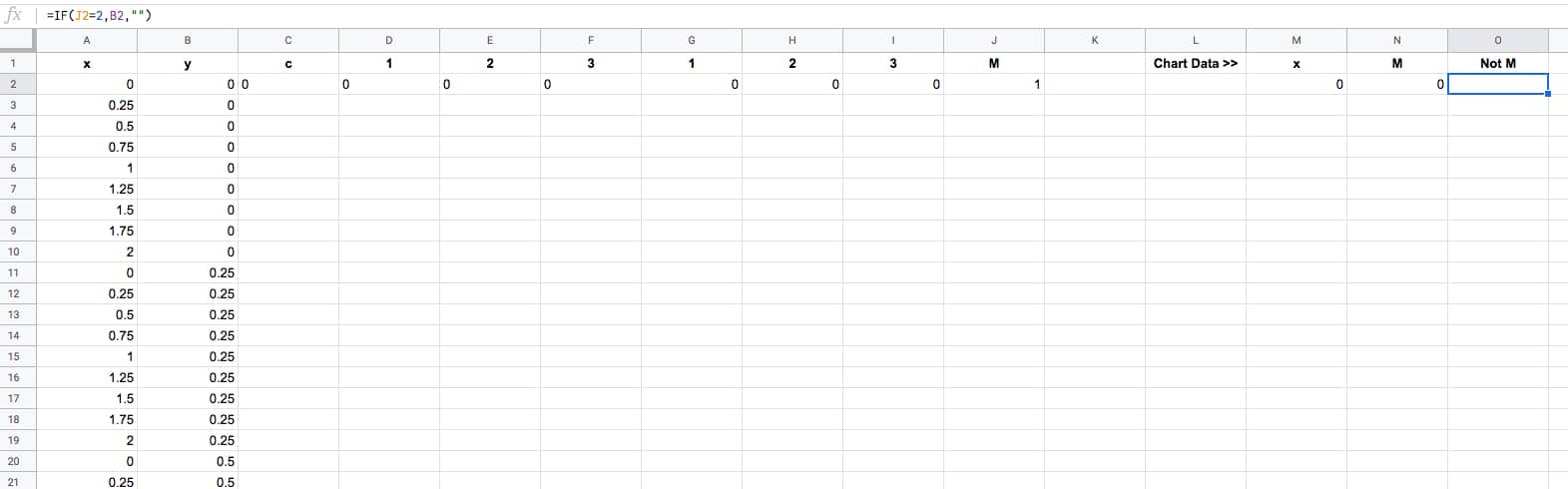The final task is to drag that first row of formulas down to the bottom of the dataset, so that every row is filled in with the formulas (click to enlarge):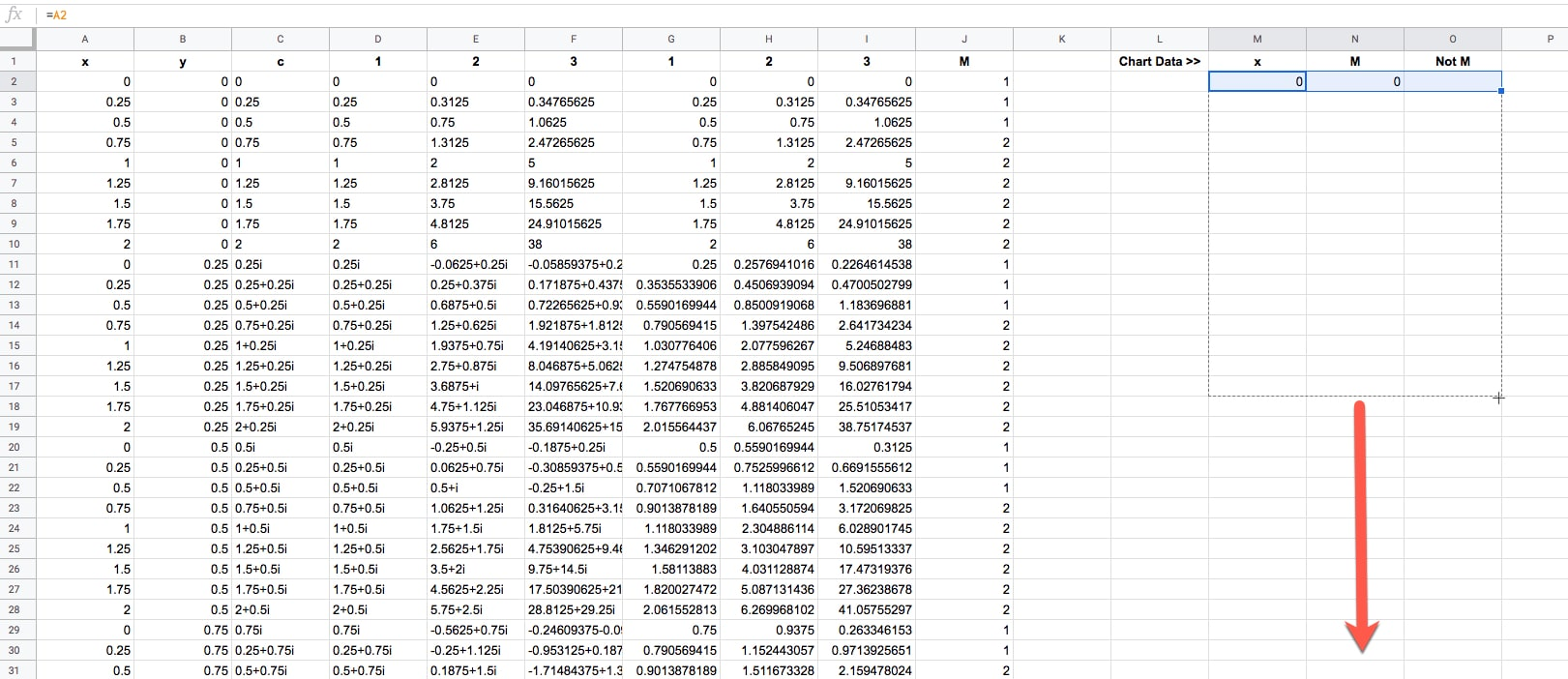Ok, now we're ready to draw some pretty pictures!

### Drawing The Mandelbrot Set In Google Sheets

Highlight columns M, N and O in your dataset.

Click Insert > Chart.

It'll open to a weird looking default Column Chart, so change that to a Scatter chart under Setup > Chart type.

Change the series colors if you want to make the Mandelbrot set black and the non-Mandelbrot set some other color. I chose light blue.

And finally, resize your chart into a square shape, using the black grab handles on the borders of the chart object.

Boom! There it is in all it's glory:Note: you may need to manually set the min and max values of the horizontal and vertical axes to be -2 and 2 respectively in the Customize menu of the chart editor.

## How To Draw A Better MandelBrot Set in Google Sheets

### Generating Data Automatically

Generating those x-y coordinates manually is extremely tedious and not really practical beyond the simple example above.

Thankfully you can use the awesome SEQUENCE function in Google Sheets to help you out. This formula will generate the x-y coordinates for a 33 by 33 grid, which gives a more filled in image than the simple example above.

```=ArrayFormula(UNIQUE({ MOD(SEQUENCE(289,1,0,1),17)/8, ROUNDDOWN(SEQUENCE(289,1,0,1)/17)/8; -MOD(SEQUENCE(289,1,0,1),17)/8, ROUNDDOWN(SEQUENCE(289,1,0,1)/17)/8; MOD(SEQUENCE(289,1,0,1),17)/8, -ROUNDDOWN(SEQUENCE(289,1,0,1)/17)/8; -MOD(SEQUENCE(289,1,0,1),17)/8, -ROUNDDOWN(SEQUENCE(289,1,0,1)/17)/8 }))```

Then drag the other formulas down your rows to complete the dataset, in the same way we did above.

Then you can highlight columns M, N and O to draw your chart again.

Note: you may need to manually set the min and max values of the horizontal and vertical axes to be -2 and 2 respectively in the Customize menu of the chart editor.

With three iterations, the chart looks like: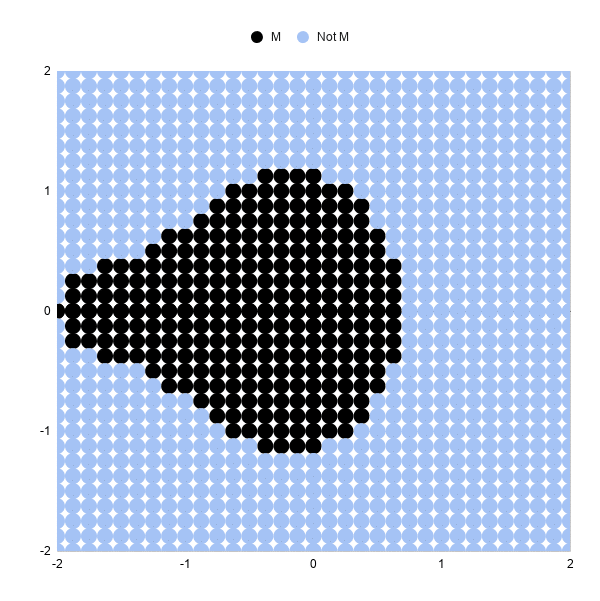### Increasing The Iterations

Let's look at 5 iterations and 10 iterations, and you'll see how much detail this adds.

To move from 3 iterations to 5, we need to add some columns to our Sheet and repeat the algorithm two more times.

So, insert two blank columns between F and G. Label the headings 4 and 5.

Drag the the formula in F2 across the new blank columns G and H (this is the z² + c equation as a Google Sheet formula):

In G2:

`=IMSUM(IMPOWER(F2,2),\$C2)`

In H2:

`=IMSUM(IMPOWER(G2,2),\$C2)`

We need to add the corresponding size calculation columns. Between K and L, insert two new blank columns and drag the IMABS formula across.

Now in L2:

`=IMABS(G2)`

And in M2:

`=IMABS(H2)`

Finally, update the IF statement in column to ensure it's testing the value in column M now:

`=IFERROR(IF(M2<=2,1,2),2)`

And that's it!

Our chart should update and look like this: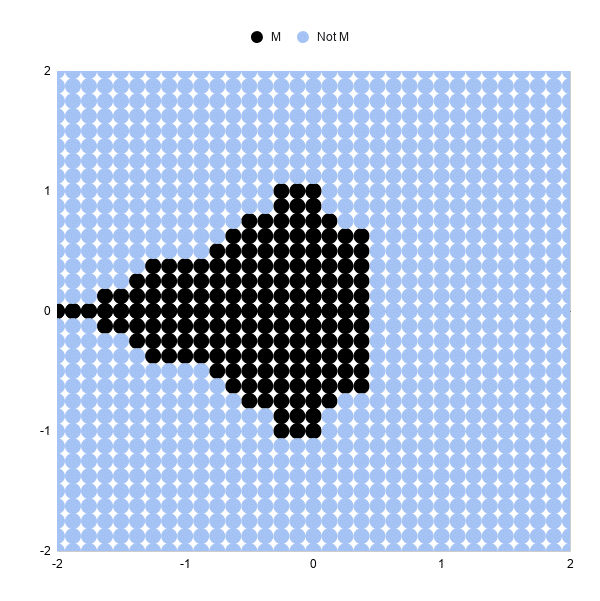You can see the shape of the Mandelbrot set much more clearly now.

Increasing from 5 iterations to 10 iterations is exactly the same. Add 5 blank columns and populate with the formulas again.

The resulting 10 iteration chart is better again: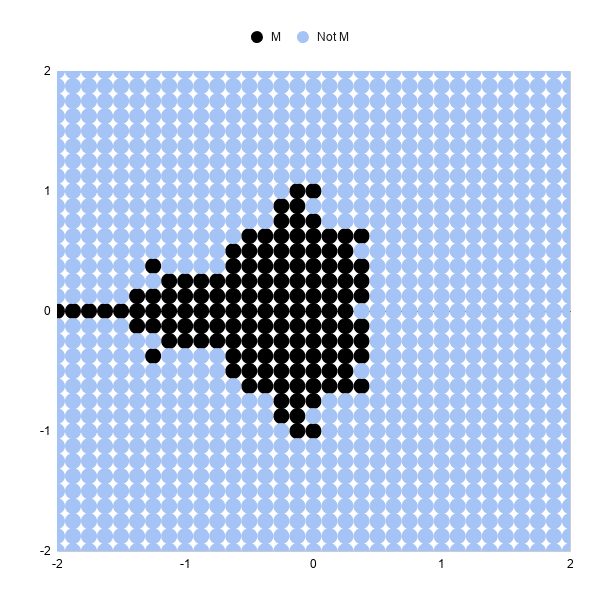### Increasing The Number Of Data Points

Increasing the number of points to 6,561 in an 81 by 81 grid will give a more "complete" picture than the examples above.

This sequence formula will generate these datapoints:

```=ArrayFormula(UNIQUE({ MOD(SEQUENCE(1681,1,0,1),41)/20, ROUNDDOWN(SEQUENCE(1681,1,0,1)/41)/20; -MOD(SEQUENCE(1681,1,0,1),41)/20, ROUNDDOWN(SEQUENCE(1681,1,0,1)/41)/20; MOD(SEQUENCE(1681,1,0,1),41)/20, -ROUNDDOWN(SEQUENCE(1681,1,0,1)/41)/20; -MOD(SEQUENCE(1681,1,0,1),41)/20, -ROUNDDOWN(SEQUENCE(1681,1,0,1)/41)/20 }))```

Be warned, as you increase the number of formulas in your Sheet and the number of points to plot in your scatter chart, your Sheet will start to slow down!

We can add color bands to show which iteration a given point "escaped" towards infinity.

For example, all of the points that are larger than our threshold on iteration 5 get a different color than those that are less than the threshold at iteration 5, but become larger on iteration 6.

The formulas for the iterations and size are the same as the examples above.

Then I determine if the point is still in the Mandelbrot set:

`=IF(ISERROR(AG2),"No",IF(AG2<=2,"Yes","No"))`

And then what iteration it "escapes" to infinity, or beyond the threshold of 2 in this example:

`=IF(AH2="Yes",0,MATCH(2,S2:AG2,1))`

These formulas are shown in the Google Sheets template:

Next we create the series for the chart:

First, the Mandelbrot set:

`=IF(AH2="Yes",B2,"")`

Then series 1 to 15:

`=IF(\$AI2=AK\$1,\$B2,"")`

The range for the scatter plot is then:

`A1:A6562,AJ1:AY6562`

where column A is the x-axis values and columns AJ to AY are the y-axis series.

Drawing the scatter plot and adjusting the series colors results in a pretty picture (this is an 81 by 81 grid):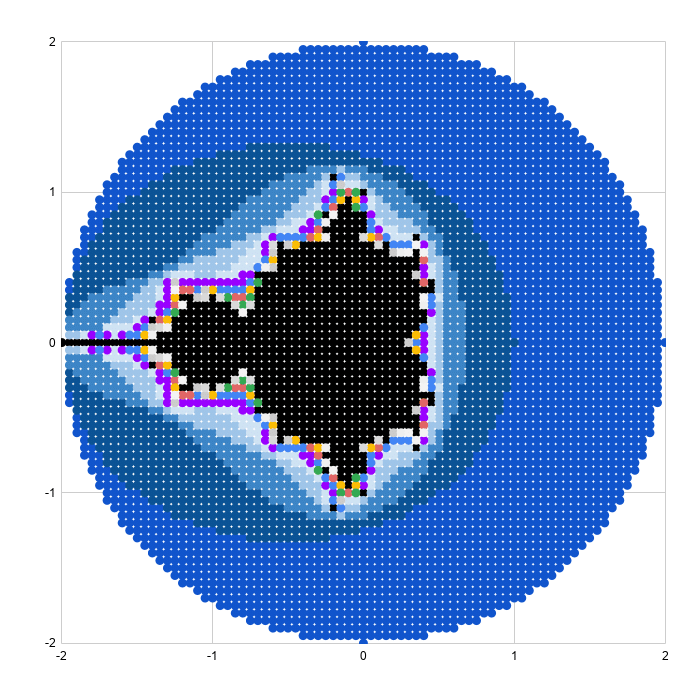### Reaching Google Sheets Practical Limit

As a final exercise, I increased the plot size to 40,401 representing a grid of 201 by 201 points. This really slowed the sheet down and took about half an hour to render the scatter plot, so not something I'd recommend.

The picture is very pretty though!The 40,401 x-y coordinates can be generated with this array formula:

```=ArrayFormula(UNIQUE({ MOD(SEQUENCE(10201,1,0,1),101)/50, ROUNDDOWN(SEQUENCE(10201,1,0,1)/101)/50; -MOD(SEQUENCE(10201,1,0,1),101)/50, ROUNDDOWN(SEQUENCE(10201,1,0,1)/101)/50; MOD(SEQUENCE(10201,1,0,1),101)/50, -ROUNDDOWN(SEQUENCE(10201,1,0,1)/101)/50; -MOD(SEQUENCE(10201,1,0,1),101)/50, -ROUNDDOWN(SEQUENCE(10201,1,0,1)/101)/50 }))```

## Zooming In On The Mandelbrot Set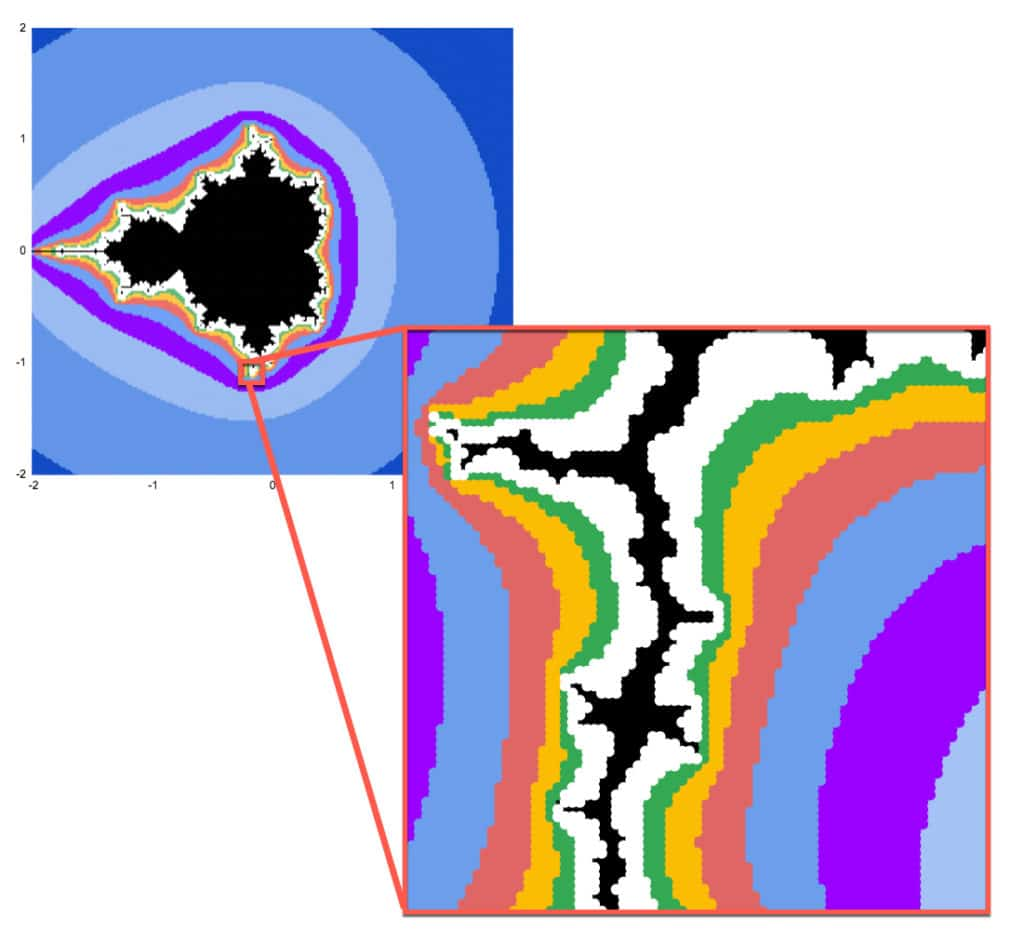Mandelbrot sets have the property of self-similarity.

That is, we can zoom in on any section of the chart and see the same fractal geometry playing out on infinitely smaller scales. This is but one of the astonishing properties of the Mandelbrot set.

Google Sheets is definitely not the best tool for exploring the Mandelbrot set at increasing resolution. It's too slow to render graphically and too manual to make the changes to the formulas and axis bounds.

However, as the maxim says: the best tool is the one you have to hand.

So, if you want to explore in Google Sheets it is possible:

### Generating Zoomed Data

I'm going to zoom in on the point: -0.17033700000, -1.06506000000

(Thanks to this article, The Mandelbrot at a Glance by Paul Bourke, which highlighted some interesting points to explore.)

Starting with this formula in cell A2 to generate the 6,561 data points (I wouldn't recommend going above this because it becomes too slow):

```=ArrayFormula(UNIQUE({ MOD(SEQUENCE(1681,1,0,1),41)/20, ROUNDDOWN(SEQUENCE(1681,1,0,1)/41)/20; -MOD(SEQUENCE(1681,1,0,1),41)/20, ROUNDDOWN(SEQUENCE(1681,1,0,1)/41)/20; MOD(SEQUENCE(1681,1,0,1),41)/20, -ROUNDDOWN(SEQUENCE(1681,1,0,1)/41)/20; -MOD(SEQUENCE(1681,1,0,1),41)/20, -ROUNDDOWN(SEQUENCE(1681,1,0,1)/41)/20 }))```

In columns C and D, I transformed this data by change the 0,0 center to -0.17033700000, -1.06506000000 and then adding the values from A and B to C and D respectively, divided by 100 to zoom in.

`=C\$2+A3/100`
`=D\$2+B3/100`

The rest of the process is identical.

I set the chart axes min and max values to match the min and max values in each of column C (x axis) and D (y axis).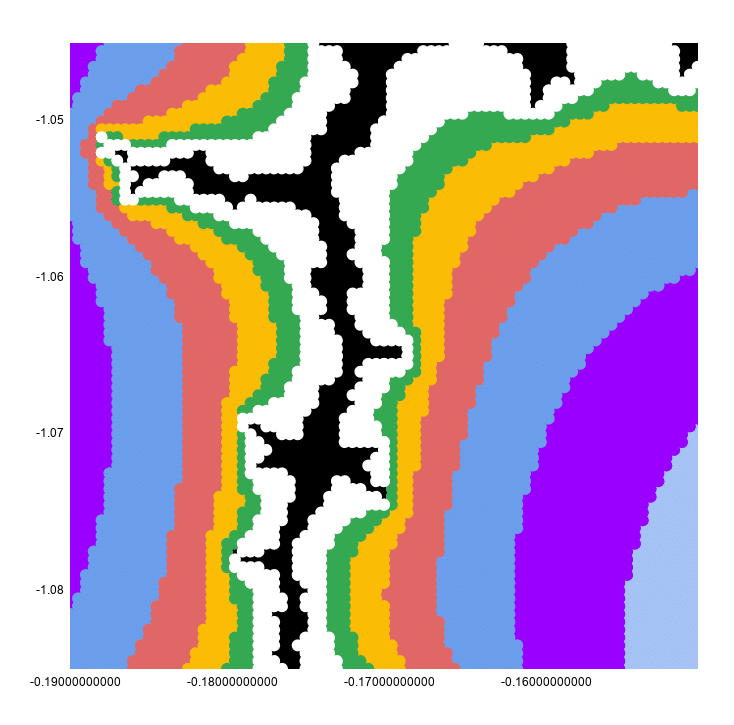This looks continuous because the chart has a point size of 10px to make it look better.

If I reset that to 2px, you can see clearly that this is still a scatter plot: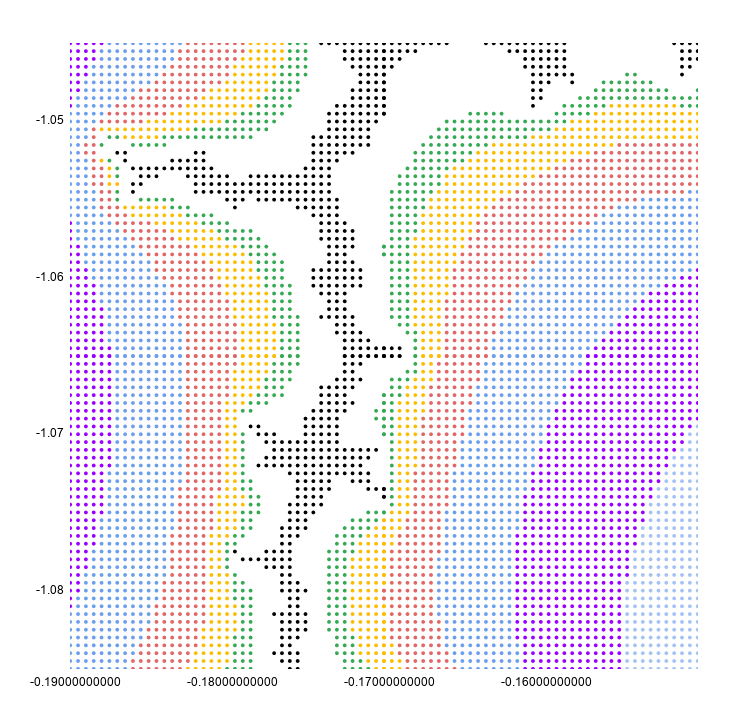I hope you enjoyed that exploration of the Mandelbrot set in Google Sheets! Let me know your thoughts in the comments below.

## Complex Numbers in Google Sheets

Complex numbers are numbers in the form a + bi, where i is the square root of -1.

What on earth does that mean?

## Imaginary And Complex Numbers

To start, consider an integer, say the number 4. The square root of 4 is 2. Easy peasy.

Now consider -4. What’s the square root of that?

It’s not -2, because -2 * -2 = 4 (a minus multiplied by a minus is a positive in mathematics).

No real number will equal the square root of – 4, so we need a new number.

This new number is called an imaginary number (no, I didn’t just make that up!) and is denoted by i and defined as the square root of -1.

`i = √-1`

or put another way:

`i² = -1`

A complex number is a number that has real and imaginary parts, and can be expressed in the form:

`a + bi`

where a and b are real numbers and i is the imaginary number defined above.

We can look at complex numbers visually in a 2-dimensional plane, with the x-axis representing the real numbers and the y-axis representing the imaginary numbers: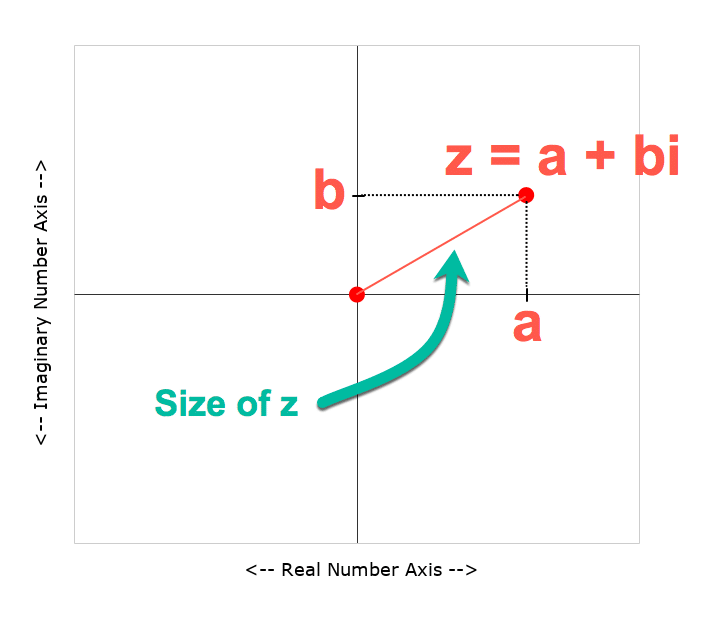Complex numbers are usually represented by the letter z, and written:

`z = a + bi`

Let’s go and create one in Google Sheets!

## Complex Numbers In Google Sheets

Let’s create a complex number in Google Sheets.

Starting in a blank Sheet, add the numbers 3 and 2 into cells A1 and B1 respectively.

In C1, add this formula with the COMPLEX function:

`=COMPLEX(A1,B1,"i")`

This is what our complex number looks like in Google Sheets: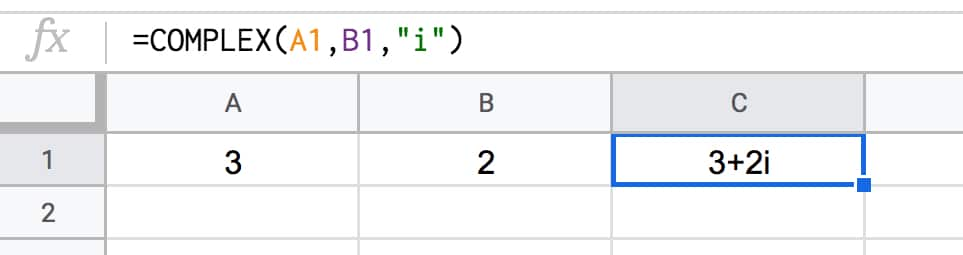### Size Of A Complex Number

We can calculate the size of a complex number, called its absolute value or modulus value and written |z|, by measuring how far it is from the center point (0,0).

We do this using the Pythagorean formula for the longest side of a right angle triangle, namely the square root of the squares of the other two sides.

Google Sheets has a handy function, called IMABS, that will calculate this value for us.

Put this formula into cell D1:

`=IMABS(C1)`

It gives the result 3.605551275.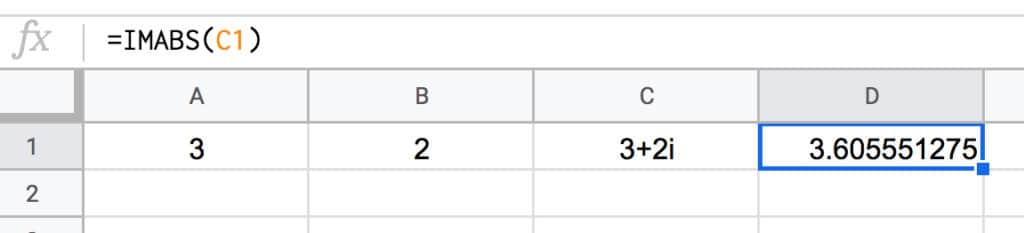This is the same as doing the square root of the squares of the other two sides:

`=SQRT(3^2+2^2)`

The next operation we need to know how to do with complex numbers is how to add them.

What’s 3 + 2i added to 5 + 7i?

To get the answer we add the real parts and imaginary parts separately, and combine into a new complex number.

So

```z = (3 + 2i) + (2 + 7i) = (3 + 2) + (2i + 7i) = 5 + 9i```

Back in our Google Sheet, I’ve added a new row for the new complex number and used the IMSUM function to add them together:

`=IMSUM(C1:C2)`

which looks like this in our Google Sheet: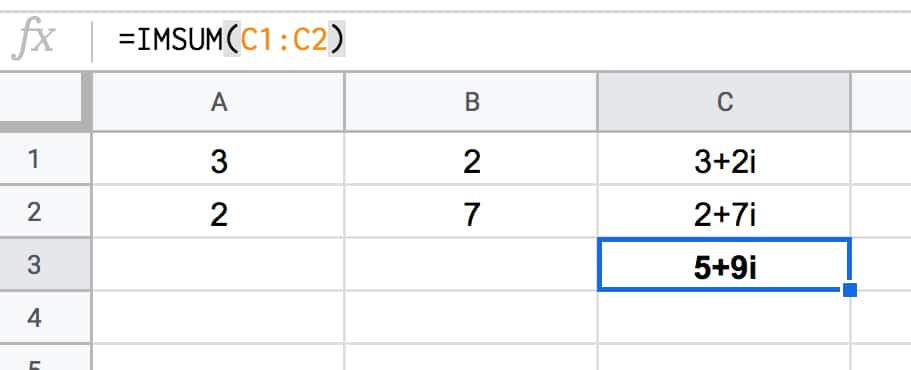### Squaring Complex Numbers

Another operation we need to perform on our complex numbers is to square them.

The IMPOWER function does the trick. It returns a complex number raised to a power.

In our Google Sheet, use this formula in cell D1:

`=IMPOWER(C1,2)`

which gives the answer 5 + 12i, calculated as follows:

```(3 + 2i)² = (3 + 2i) * (3 + 2i) = 3 * 3 + 2i * 3 + 3 * 2i + 2i * 2i = 9 + 6i + 6i + 4 * i² = 9 + 12i + 4 * -1 = 9 - 4 + 12i = 5 + 12i```

### Extracting Real And Imaginary Parts

You can use the IMREAL function to return the real coefficient of a complex number (the “a” part).

And IMAGINARY function returns the imaginary coefficient (the “b” part).

There are other, more specialized complex numbers functions in Google Sheets too, but they’re beyond the scope of this article.

## Why Are Complex Numbers Useful?

For mathematicians, complex numbers are just as “real” as the real numbers.

They have applications in many areas of mathematics, physics and engineering.

One area where you may have seen them without realizing, is in fractal geometry and specifically pictures of a beautiful set of complex numbers called the Mandelbrot set:## Formula Challenge #4: Sort A Column By Last Name

This Formula Challenge originally appeared as Tip #108 of my weekly Google Sheets Tips newsletter, on 29 June 2020. Sign up so you don’t miss future Formula Challenges!

Find all the Formula Challenges archived here.

## The Challenge: Sort A Column By Last Name

Start with a list of any ten two-word names in column A, like so: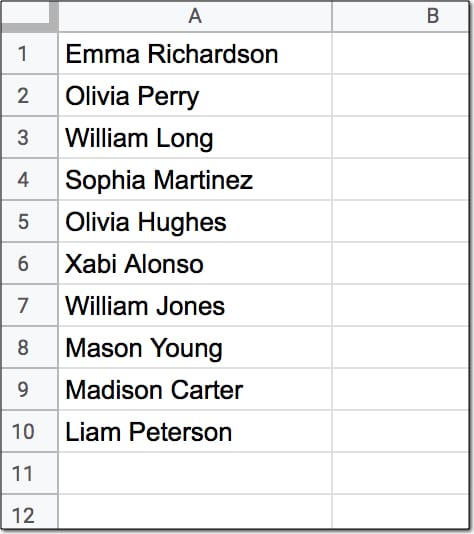Your challenge is to create a single formula in cell B1 (shown in yellow below) that sorts this list alphabetically by the last name, like this: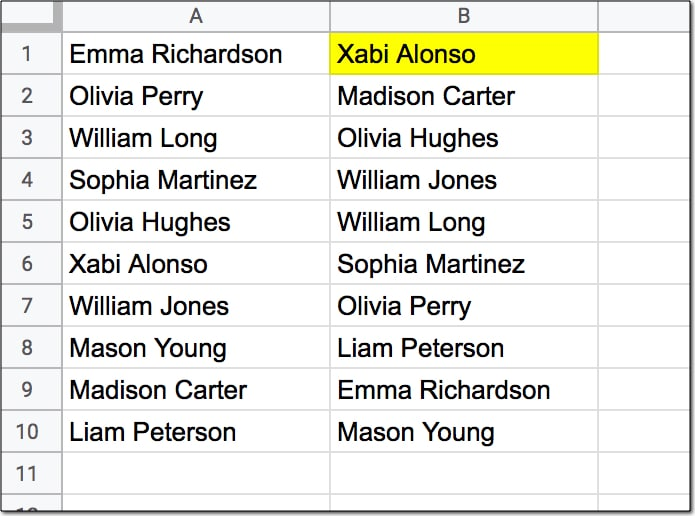Assumptions:

• The names to sort are in the range A1:A10
• Each name consists of a first name and last name only, with a single space between them
• None of the names have prefixes or suffixes
• The formula in cell B1 should output the full names in range B1:B10

## Solutions To Sort A Column By Last Name

I received over 90 replies to this formula challenge with at least 10 different methods for solving it. This was super interesting to see!

Congratulations to everyone who took part.

I learnt so much from the different replies, many of which proffered shorter and more elegant solutions than my own original formula.

Here I present the best 4 solutions, chosen for their simplicity and elegance.

There’s a lot to learn by looking through them.

### 1. SORT/INDEX/SPLIT method

Solution:

Use this formula in cell B1 to sort the data alphabetically by the last name:

`=SORT(A1:A10,INDEX(SPLIT(A1:A10," "),,2),1)`

This is a beautiful solution.

It’s deceptively simple, as you’ll see below when we break it down. And yet, only a handful of people submitted this solution.

How does this formula work?

Let’s use the onion method to peel back the layers and build this formula in steps from the innermost layer.

Step 1:

Enter this formula as a starting point:

`=SPLIT(A1:A10," ")`

It takes the range A1:A10 as an input and splits the names on the space character. That works in this example because of our rigid assumptions that all names are two-word names.

The output is a single row with the name split across two cells. (Adding an ArrayFormula would give an output of all ten names, but it’s not required when we plug this split into the INDEX function.)

Step 2:

Wrap the formula from step 1 in an INDEX function:

`=INDEX(SPLIT(A1:A10," "),,2)`

Leave the row argument (the second argument) in the function blank and choose column 2 in the column argument (the third one). This formula outputs the list of second names, still in the original order however.

Step 3:

The SORT function takes three arguments: the range to sort, the column to sort on, and whether to sort ascending or descending.

So A1:A10, the range containing the full names, is the range we want to sort.

Then the column of last names created in step 2 is the column we want to sort on. This is our second argument.

Then the third argument is a TRUE or 1 to indicate we want to sort the names ascending.

So plugging these three arguments into the SORT function gives the following formula:

`=SORT(A1:A10,INDEX(SPLIT(A1:A10," "),,2),1)`

This formula can be modified to include the whole of column A if there are more than 10 names. Simply change the range from A1:A10 to A1:A as follows:

`=SORT(A1:A,INDEX(SPLIT(A1:A," "),,2),1)`

### 2. QUERY method

Solution:

Use this formula in cell B1 to sort the data alphabetically by the last name:

`=ArrayFormula(QUERY({A1:A10,SPLIT(A1:A10," ")},"select Col1 order by Col3"))`

Step 1:

Step 1 is the same as the step 1 in solution 1 above, and uses the SPLIT to separate the names into first and last names in separate columns:

`=SPLIT(A1:A10," ")`

Step 2:

Step 2 combines these two split columns with the original column of names using curly braces to construct an array of full name, first name and last name.

`=ArrayFormula({A1:A10,SPLIT(A1:A10," ")})`

The ArrayFormula wrapper is necessary to output all 10 values in this example.

Step 3:

The array created by step 2 is used as the input data range in the QUERY function in step 3:

`=ArrayFormula(QUERY({A1:A10,SPLIT(A1:A10," ")},"select Col1 order by Col3"))`

Since the input range of the QUERY function is constructed with the curly brace notation {…} we are required to use the Col1, Col2, etc. notation to access the columns. The query clause is pretty simple. Select only column 1 of the array (the full name) but sort by column 3 (the last name).

Note, the ArrayFormula can be brought outside the QUERY to be the outer wrapper.

This formula can be extended easily to the whole of column A by changing the range to A1:A and adding a WHERE clause to filter out the blank rows:

`=ArrayFormula(QUERY({A1:A,SPLIT(A1:A," ")},"select Col1 where Col1 is not null order by Col3"))`

### 3. REGEX method I

Solution:

Use this formula in cell B1 to sort the data alphabetically by the last name:

`=INDEX(SORT(REGEXEXTRACT(A1:A10,"((.*)( .*))"),3,1),,1)`

If the first solution was the shortest and simplest, then this is perhaps the most elegant.

It only references the data range A1:A10 once, whereas all the other solutions presented here reference the data range twice.

Step 1:

Use the REGEXEXTRACT function to split the data into three columns of full name, first name and last name:

`=REGEXEXTRACT(A1:A10,"((.*)( .*))")`

The REGEX functions in Google Sheets use the re2 flavor of regex.

This formula uses numbered capturing groups to capture the data, denoted by (.*) and ( .*) with the second having a space. The .* simply says match zero or more of any character.

In other words the (.*)( .*) construction separates into first and last names. Adding another matching group with the additional parentheses ((.*)( .*)) also matches the full name.

These three columns are passed into the SORT function in STEP 2:

Step 2:

`=SORT(REGEXEXTRACT(A1:A10,"((.*)( .*))"),3,1)`

The data is sorted on column 3 containing the last name. The 1 represents a TRUE value which sorts the data ascending from A-Z.

Step 3:

In the final step, we use the INDEX function to return only the first column, which has the full name.

`=INDEX(SORT(REGEXEXTRACT(A1:A10,"((.*)( .*))"),3,1),,1)`

### 4. REGEX method II

Solution:

Use this formula in cell B1 to sort the data alphabetically by the last name:

`=SORT(A1:A10,REGEXEXTRACT(A1:A10,"(?: )(\w*)"),1)`

This is the same method as the first solution, but uses REGEXEXTRACT instead of INDEX/SPLIT. So it’s one less function, but the REGEXEXTRACT function is much harder to understand than the INDEX/SPLIT combination for those unfamiliar with the black magic of regular expressions.

Step 1:

Use the REGEXEXTRACT function to extract the last names from the data:

`=REGEXEXTRACT(A1:A10,"(?: )(\w*)")`

The (?: ) is a non-capturing group matching the space. In other words the REGEXEXTRACT matches the space but doesn’t extract it.

The \w character matches word characters (≡ [0-9A-Za-z_]) and the * means match zero or more word characters but prefer more.

The parentheses ( ) around the \w* make it a captured group so it’s extracted.

See, regular expressions are as clear as mud.

Ok, so what’s happening is that it matches on the space, but doesn’t return it. Then it matches on the word group that follows the space and returns that. Hence we get an output range of last names only.

Step 2:

The final step is to sort range A1:A10 using the last names extracted in step 2, and sort them ascending. This step is identical to the implementation in solution 1.

`=SORT(A1:A10,REGEXEXTRACT(A1:A10,"(?: )(\w*)"),1)`

This formula can be modified to include the whole of column A if there are more than 10 names. Simply change the range from A1:A10 to A1:A as follows:

`=SORT(A1:A,REGEXEXTRACT(A1:A,"(?: )(\w*)"),1)`

There we go!

Four brilliant solutions to an interesting formula challenge.

## Connected Sheets: Analyze Big Data In Google Sheets

The future of Google Sheets is analyzing millions, even billions, of rows of data with regular formulas and pivot tables.

The future of Google Sheets is Connected Sheets.

That’s a bold claim, so let’s explore it.

Check out this formula operating on 1.5 billion rows of data:I just ran a COUNT function across 1.5 Billion rows of data. Inside my Google Sheet.

1.5 Billion rows of data!

Whaaaaat?

Fzzzzzzt……that’s the sound of all the circuit boards in my head frying simultaneously 🤯

## Connected Sheets

I think we can all agree there’s a lot of data out there. We live in a data-rich world. And it’s only ever going to grow from here as more and more people and devices come online.

And we can also agree that “big data” is too large to store and analyze in your Google Sheets.

Until now…

Connected Sheets is a new data feature in Google Sheets for customers of G Suite Enterprise, G Suite Enterprise Essentials and G Suite Enterprise for Education.

You use the Connected Sheets feature to analyze millions or billions of rows of data inside your Google Sheets, using regular functions, pivot tables and charts.

The data lives in Google BigQuery, which is a big data analysis product from Google Cloud.

BigQuery is an incredible tool but does require knowledge of SQL code to use it.

Connected Sheets circumvents that need and gives Google Sheets users the ability to analyze big data inside their Sheets, using the familiar interface.

## What Can Connected Sheets Do?

Connected Sheets can do similar operations with big datasets that you’re used to doing with regular datasets in Google Sheets, namely:

1. Running a defined set of functions on big datasets*
2. Creating calculated columns with big datasets*
3. Extracting small data tables, called Extracts, from big datasets*, which can be used like regular Google Sheets tables
4. Creating pivot tables from big datasets*
5. Creating charts from big datasets*
6. Scheduling automatic data refresh jobs to keep data current

* i.e. tables with millions or even billions of rows of data in BigQuery

I think the best way to explore these features is to run through a quick demo data analysis project.

## Analysis Of New York Citibike Data

You need to have a project setup in BigQuery with billing enabled before you can connect to it from the Connected Sheets menu.

When you create a new Connected Sheet, you’re prompted through a series of options to select a BigQuery project and table that you want to connect to.

In this example, I’ve connected to the New York Citibike public dataset, which has 59 million rows of data and 16 columns, which would be nearly a billion cells of data if we could put it into a spreadsheet.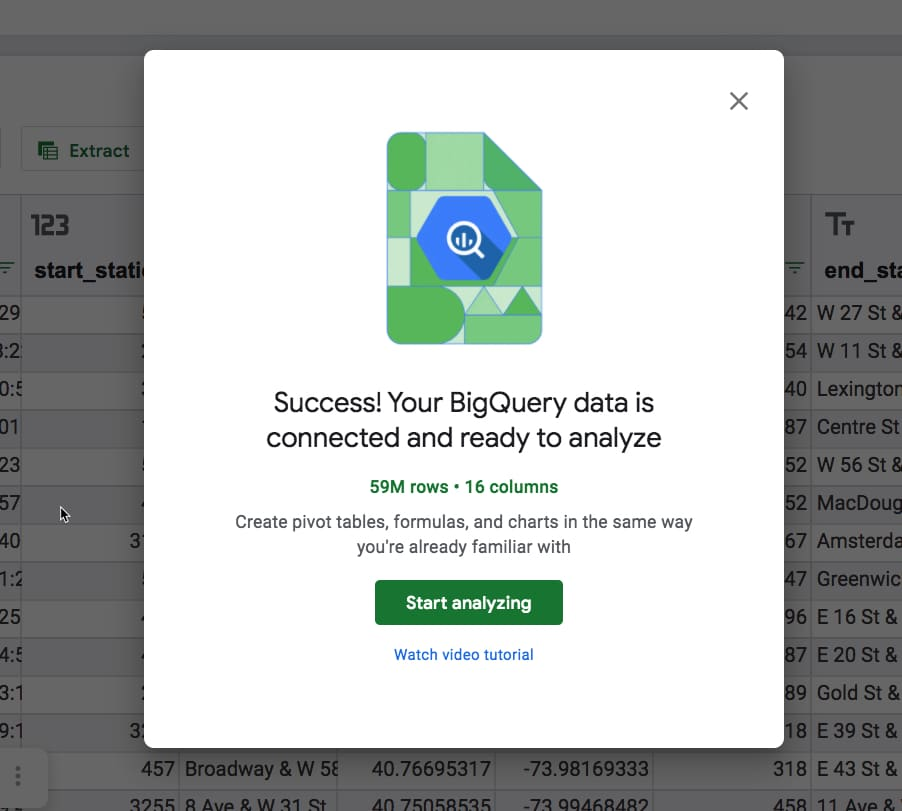When it opens, you’re shown a preview of the dataset, i.e. all of the columns and the first 500 rows.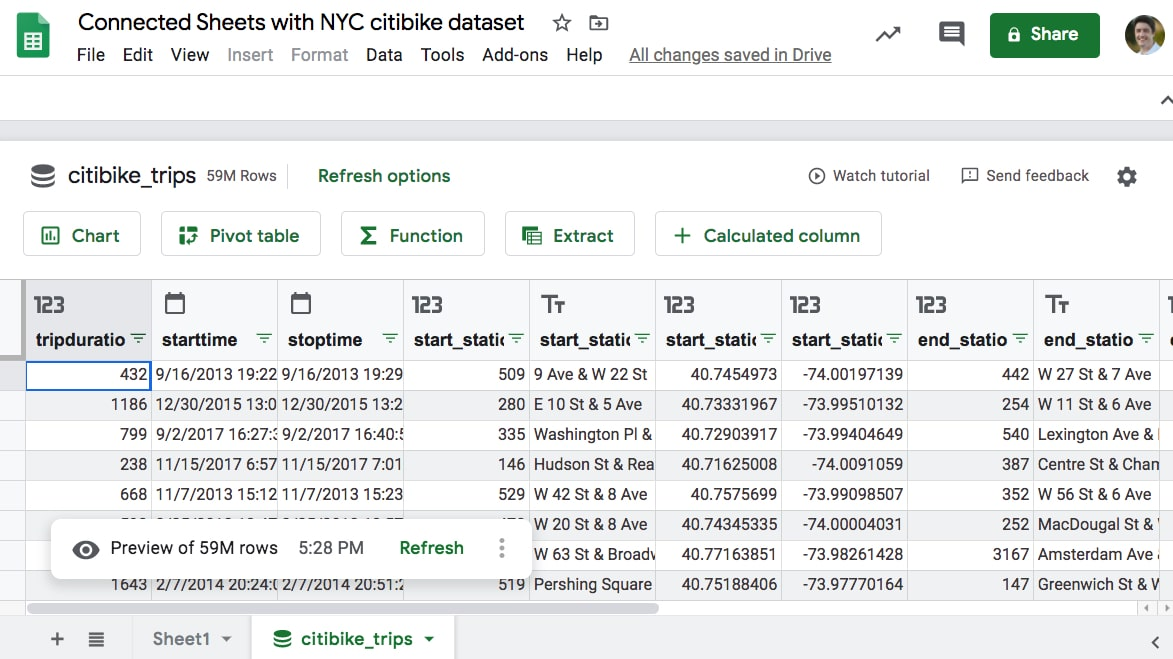It looks like an ordinary Google Sheet, but there are five action buttons above the Sheet: Chart, Pivot Table, Function, Extract and Calculated Column.

You’ll also notice the word “Refresh options” next to the table name and “Refresh” down at the bottom in the preview note. You need to refresh periodically to include any new data from BigQuery in your Connected Sheets results. You can schedule these refreshes to happen automatically.

Now let’s explore each of the features in turn, starting with functions:

## Functions with Connected Sheets

Functions in Connected Sheets are useful for getting specific answers from your data, i.e in situations when you know what you’re looking for.

For example, if I want to count how many rows of data there are in a dataset then I would use a simple COUNT function.

It’s exactly the same here with the Big Dataset in the Connected Sheet. I use a simple function in Connected Sheets.

The flow is a little different, as there is an extra click to activate the function, but otherwise it’s a regular ole’ formula: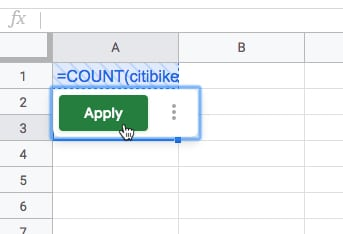The formula looks like this in the Sheet:

`=COUNT(citibike_trips!starttime)`

`53,108,721`

53 million rows of data, each representing a bike trip with one of New York City’s Citibike bikes.

Boom! It’s as easy as that!

Comparison: To do the same calculation in BigQuery, you need to write a simple query in the BigQuery editor:

```SELECT
COUNT(starttime)
FROM
`bigquery-public-data.new_york_citibike.citibike_trips````

Which gives the same answer of `53,108,721`

As you can see, it requires knowing your way around BigQuery and of course, how to write basic SQL.

Wait a minute though!

This dataset is supposed to have 59m rows of data, not 53m.

There must be some blank rows (null values) in the data.

Let’s use Connected Sheets to test that hypothesis, using the Extract feature.

## Connected Sheets Extracts

The Extract feature lets you extract smaller tables from the larger BigQuery dataset (remember the data shown in the Sheet is just a preview and the actual dataset lives in BigQuery).

Extracts are useful for things like extracting all records pertaining to a certain customer, or looking at the largest X transactions for example. Another use case might be investigating issues with datasets.

For example, I have a strong hunch there are null values (blanks) in the dataset.

So I create an Extract using a filter set to “Value is empty” to return a subset of empty rows.

The Extract Editor is similar to the pivot table Editor, allowing you to select which columns to include, what filters or sorts to apply and what the row limit is (how many to return).

Here’s the Extract editor prior to pressing the Apply button.

You can see the blue cross shading applied to the data area to show it hasn’t been queried yet.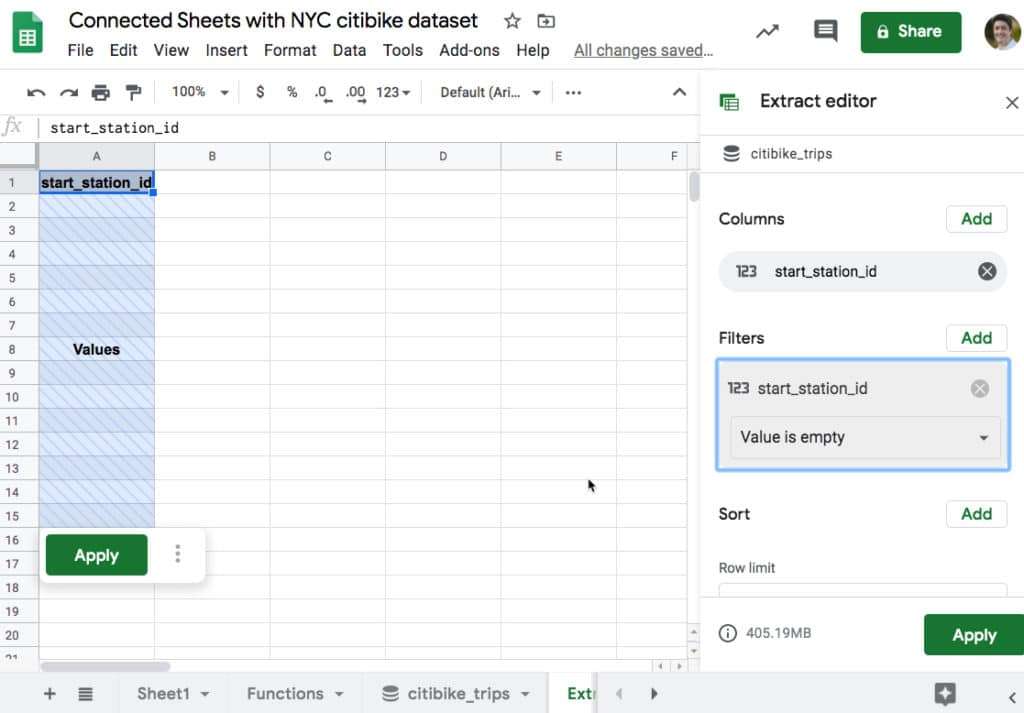With every calculation in Connected Sheets, you need to press the “Apply” button to run the query in BigQuery to return the data.

The Extract is returned with blank rows as I expected. So there are null values in the dataset!

Presumably there are about 6 million of them, but let’s confirm that using a calculated column and function in Connected Sheets to count the null values.

## Calculated Columns in Connected Sheets

Much the same way as you’d insert a column into a regular Google Sheet table and add in a formula to perform some calculation, you can do the same with Connected Sheets datasets.

Calculated columns are added to the right side of the Connected Sheets preview table (although the order doesn’t matter in a database, since you access a column by its name).

You can use a limited set of regular Google Sheets functions in calculated columns, which are then applied to the entire big dataset in BigQuery.

Let’s continue with the New York Citibike dataset.

Choose the “+ Calculated Column” button above the data preview.

The Calculated Column editor pops up, where you can enter a formula using column names instead of range references.

I’m going to multiply the start station ID by 1, because this will transform any blanks (nulls) into 0 values, which I can count.I can now create a COUNTIF function using this calculated column, to count these null rows: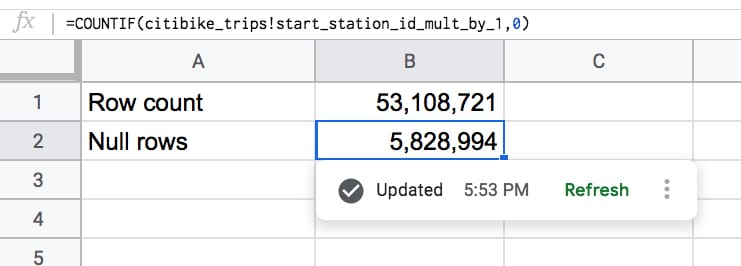Added together, those two values give us the 59 million rows in our full dataset.

Let’s add another calculated column to calculate a rider’s age.

The dataset contains the birth year for every rider, which we can use to calculate the age: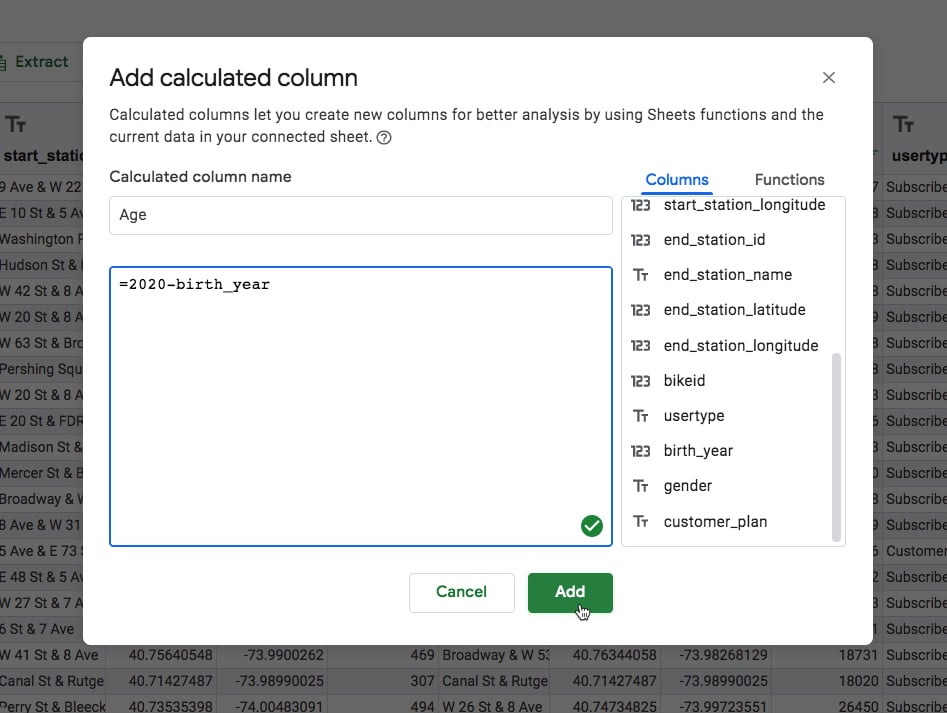There are some very old people in the data!

To me this seems unlikely (people over 100 years old riding citibikes in NYC? Hmm, I don’t know about that). These are probably errors in the data and would merit further exploration.

But for now, let’s draw a chart of rides by age.

## Connected Sheets Charts

You can showcase your results with Charts, created directly from the data in BigQuery.

Clicking on the Chart button above the dataset creates a chart and opens the chart editor.

It’s more akin to creating a pivot table than a regular Google Sheets chart. The same customization options are all there however.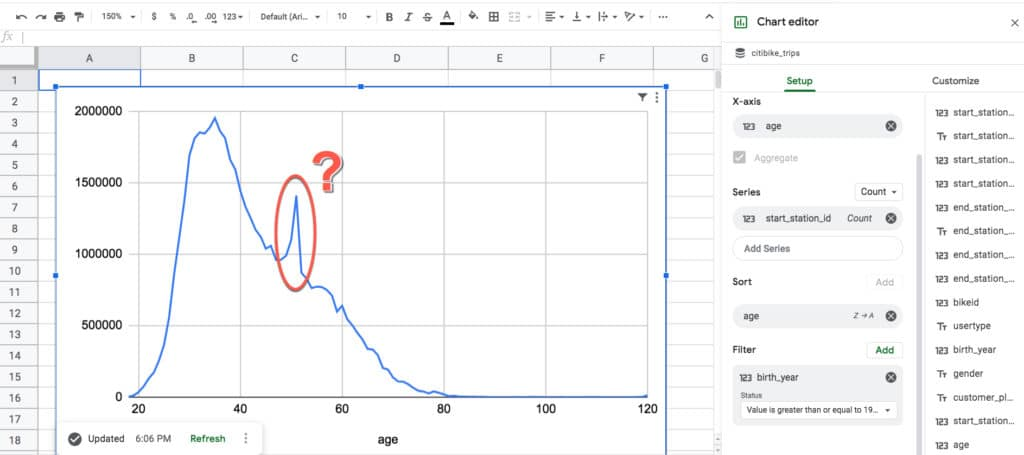Two things jumped out at me from this chart:

Firstly, it’s an example of a classic right-skewed distribution. It’s unsurprising to see the peak age of riders is in the mid- to late-thirties and then it gradually decreases from there. This is what you’d expect.

Secondly though, what on earth is that spike doing around age 51? It seems unlikely there would be a sudden, huge increase of ridership going from 50 to 51, and then equally precipitous drop-off going from 51 to 52. Perhaps it’s a strange mid-life crisis unique to New York City.

Let’s use a pivot table to investigate further.

## Connected Sheets Pivot Tables

Pivot tables are an incredible tool that allow you to explore your datasets in depth.

In essence, pivot tables aggregate (i.e. roll-up or summarize) your data.

It’s quick and easy to change the variables shown in your pivot tables, so they’re a great tool to understand what’s going on with your data.

Pivot tables in Connected Sheets condense millions or billions of rows of data into concrete insights. Insights that would be impossible to find any other way.

In this example, the pivot table shows the number of rides summarized by the age (rows) and gender (column) of each rider. It includes the full 59m rows of data.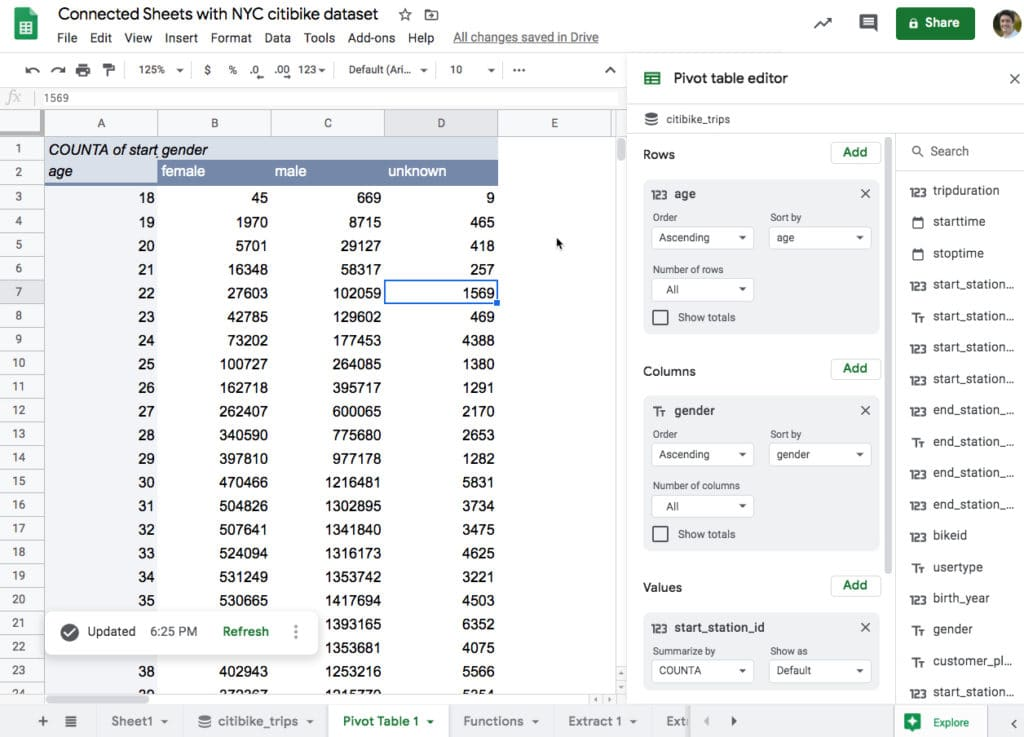Using this pivot table data, let’s recreate the rides-by-age chart again, but this time split by gender as well:

And there we go!

The mystery is revealed.

There’s a group of riders in the data whose gender is unknown and who all have the same age around 51.

Perhaps there’s a ‘prefer not to say option’ for both “How old are you?” and “Gender?” questions during the sign up process.

That would explain the unknown gender designation, but what about the spike of riders aged 51?

Well, databases use a system called Unix time or Epoch time to measure points in time. This begins on 1/1/1970. So if some riders didn’t enter a date of birth, maybe the system sets the date of birth to the start of Epoch time, causing a spike at age 51. Very interesting!

## More Connected Sheets Examples

### Proportion of round trips

Suppose we want to know how many trips are round-trips, where the bike is dropped off at the starting station?

In other words, we want to identify all rows of data where the start station and end station match.

Here’s the formula to identify the round-trip rows, which will show as TRUE values.

`= start_station_id = end_station_id`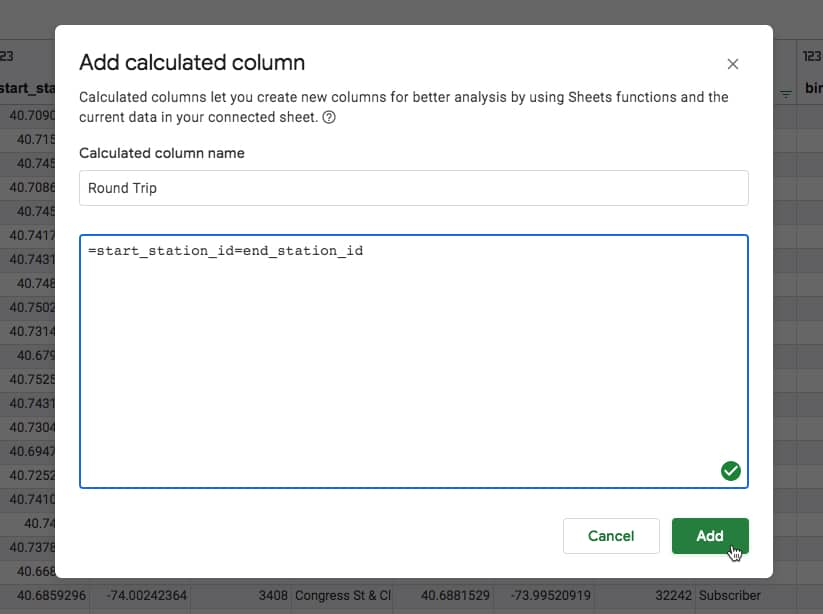What’s super cool is that we can then use these calculated columns elsewhere too, for example in a pivot table where we can now answer the original question about identifying round-trips:Out of the 59 million bike trips in the dataset, approximately 6.9 million started and finished at the same station (the TRUE values). About 12% of the trips.

### Trips per month

Here’s a chart showing the number of bike trips per month. Although this looks like a regular Google Sheets chart, it includes all 59m rows of data from BigQuery (click to enlarge):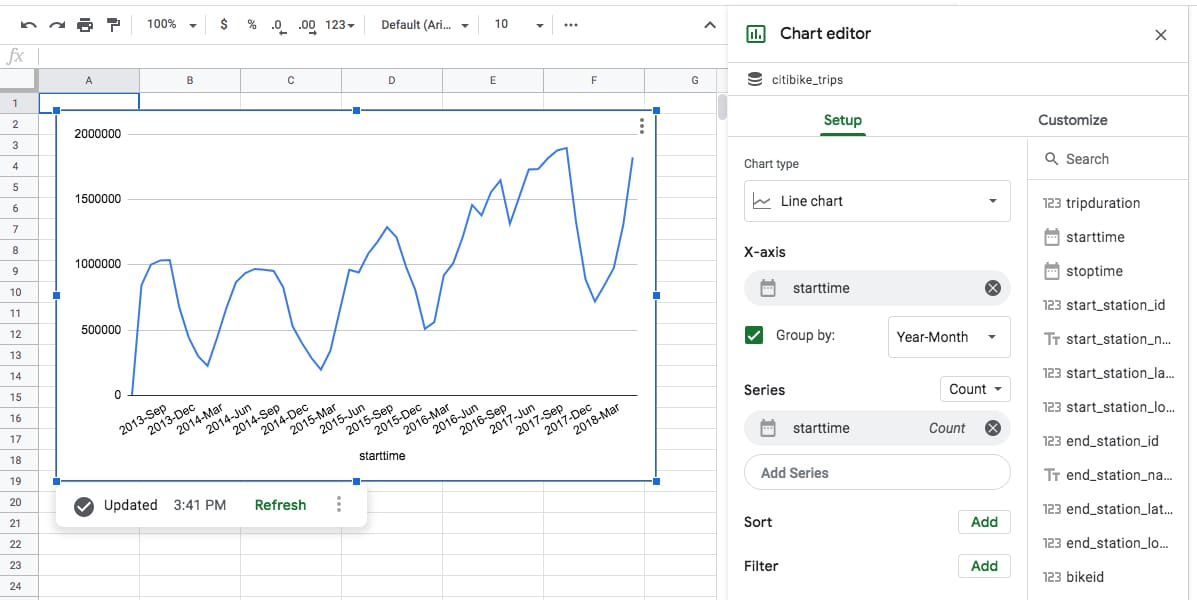It clearly shows the increasing number of trips undertaken with Citibike, as well as clear seasonal cycles (the dips in winter).

It also illustrates another important point.

The chart is a static representation of your data at the time you created it.

You can see the timestamp in the bottom left, underneath the chart.

There’s also the option to refresh the data, which updates the chart.

### Daily rides by gender

Here’s another chart example — this time drawn from the data in a Connected Sheets pivot table, rather than directly — which looks at the entire population of bike trips and counts the number on each day, split by gender.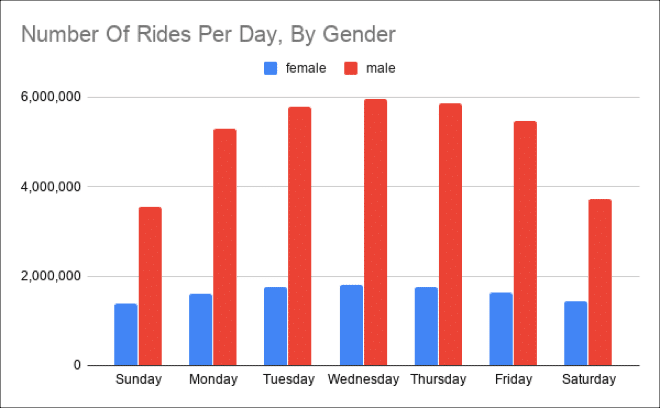It’s clear to see that the demand is driven by commuters during the week, as evidenced by the peak during the week.

It also clearly shows there have been about three times as many male riders as female riders, to date.

One final feature to showcase is the refresh pane.

## Refreshing Components

It’s important to note that the components aren’t live in the way the equivalent Google Sheets component is.

A Connected Sheets function is calculated at a given moment in time and has to be refreshed for new data to be included (whereas in a plain Google Sheet the formula will just reflect whatever data is included).

Similarly other components need to be refreshed. You can control them individually or collectively from the Refresh pane.

There’s also an option to schedule a data refresh, so that the data under consideration in your Connected Sheet is up-to-date. This can be done daily, weekly or monthly.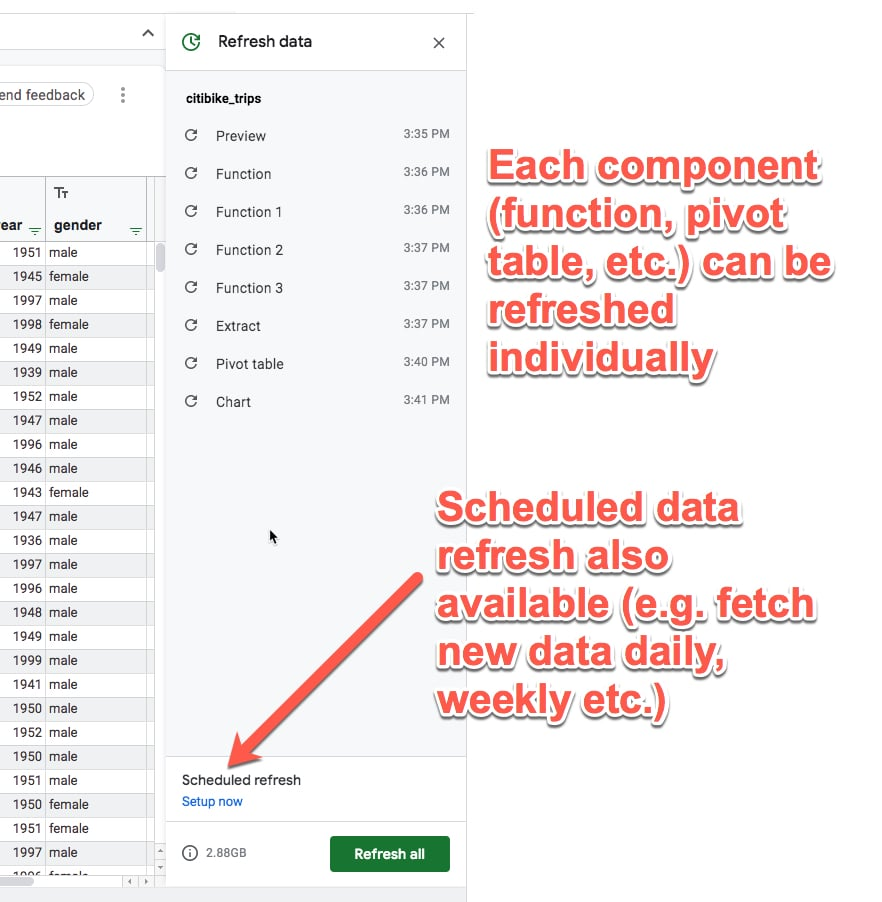## Availability of Connected Sheets

Connected Sheets is available to customers of G Suite Enterprise, G Suite Enterprise Essentials and G Suite Enterprise for Education.

A BigQuery account is required to use it and it will fall under the existing BigQuery pricing plan (prices are based on both the volume of data stored and the consumption of that data).

## Conclusion

Connected Sheets represents a huge leap forward for Google Sheets.

It opens up the world of Big Data to the regular Google Sheets user, and that is very exciting.

Through the examples in this article, I hope you’ve seen how easy it is to work with big datasets. If you can analyze small data in your Google Sheets already, then you’ll be able to apply those same skills to big datasets.

Now you won’t have to rely on a developer to write custom queries for you. You can just dive straight into the data yourself.

I can’t wait to see how this feature is used over the next few years. After all, datasets are only getting bigger from here on!

For more information, keep an eye out for announcements on the G Suite section of the Google Cloud blog and the G Suites Updates blog.

Check out this Connected Sheets YouTube tutorial from the G Suite team.

## How to import social media statistics into Google Sheets: The Import Cookbook

Google Sheets has a powerful and versatile set of IMPORT formulas that can import social media statistics.

This article looks at importing social media statistics from popular social media channels into a Google sheet, for social network analysis or social media management. If you manage a lot of different channels then you could use these techniques to set up a master view (dashboard) to display all your metrics in one place.

The formulas below are generally set up to return the number of followers (or page likes) for a given channel, but you could adapt them to return other metrics (such as follows) with a little extra work.

Caveats: these formulas occasionally stop working when the underlying website changes, but I will try to keep this post updated with working versions for the major social media statistics.

Example workbooks: Each example has a link to an associated Google Sheet workbook, so feel free to make your own copy: `File > Make a copy...`.

# How to import social media statistics into Google Sheets with formulas

##Import Facebook data

November 2018 update: The Facebook formula is working again! The trick is to use the mobile URL 😉 Thanks to reader Mark O. for this discovery.

`https://mobile.facebook.com/benlcollinsData`

or this variation of it:

`https://m.facebook.com/benlcollinsData`

Here is the formula to extract page likes:

`=INDEX(REGEXEXTRACT(REGEXEXTRACT(LOWER(INDEX(IMPORTXML(A1,"//@content"),2)),"([0-9km,.]+)(?: likes)"),"([0-9,.]+)([km]?)"),,1) * SWITCH(INDEX(REGEXEXTRACT(REGEXEXTRACT(LOWER(INDEX(IMPORTXML(A1,"//@content"),2)),"([0-9km,.]+)(?: likes)"),"([0-9,.]+)([km]?)"),,2),"k",1000,"m",1000000,1)`

The following screenshot shows these formulas: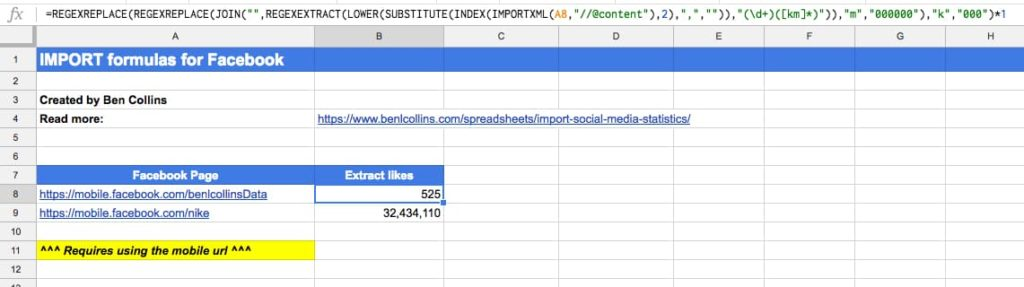##Import Twitter data

`https://mobile.twitter.com/benlcollins`

Here is the formula to extract follower count:

`=VALUE(REGEXEXTRACT(INDEX(IMPORTXML(A1,"/"),1,3),"(?:Following)(.+)(?:Followers)"))`

The following screenshot shows this formula: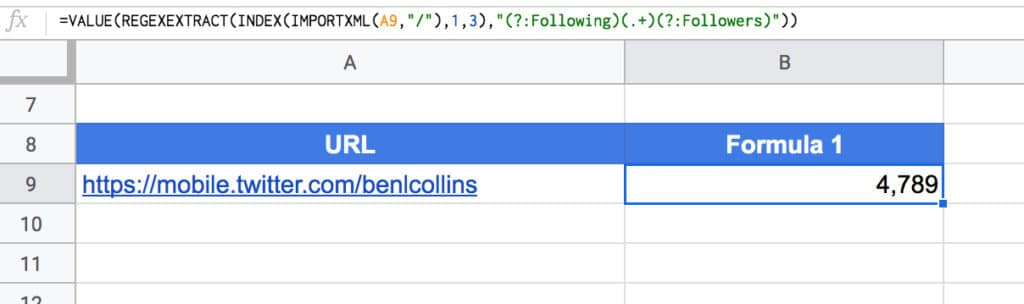Note 1: This Twitter formula seems to be particularly volatile, working fine one minute, then not at all the next. I have two Sheets open where it’ll work in one, but not the other!

^ Back to Contents

##Import Instagram data

`https://www.instagram.com/benlcollins/`

Then, this formula in cell B1 to extract the follower metadata (this may or may not work):

`=IMPORTXML(A1,"//meta[@name='description']/@content")`

This extracts the following info: “230 Followers, 259 Following, 465 Posts – See Instagram photos and videos from Ben Collins (@benlcollins)”

Next step is to combine this with REGEX to extract the followers for example. Here’s the formula to do that (still assuming url in cell A1):

`=INDEX(REGEXEXTRACT(REGEXEXTRACT(LOWER(IMPORTXML(A1,"//meta[@name='description']/@content")),"([0-9km,.]+)( followers)"),"([0-9,.]+)([km]?)"),,1) * SWITCH(INDEX(REGEXEXTRACT(REGEXEXTRACT(LOWER(IMPORTXML(A1,"//meta[@name='description']/@content")),"([0-9km,.]+)( followers)"),"([0-9,.]+)([km]?)"),,2),"k",1000,"m",1000000,1)`

This deals with any accounts that have abbreviated thousands (k) or millions (m) notations.

Alternative Approach

The following formulas to extract account metrics appear to only work for the instagram account when you are logged in.

Here’s the number of followers:

`=REGEXEXTRACT(INDEX(SPLIT(QUERY(IMPORTDATA(A1),"select Col1 limit 1 offset 181"),""""),1,2),"[\d,]+")`

Here’s the number following:

`=REGEXEXTRACT(QUERY(IMPORTDATA(A1),"select Col2 limit 1 offset 181"),"[\d,]+")`

Here’s the number of posts:

`=REGEXEXTRACT(QUERY(IMPORTDATA(A1),"select Col3 limit 1 offset 181"),"[\d,]+")`

The following screenshot shows these formulas: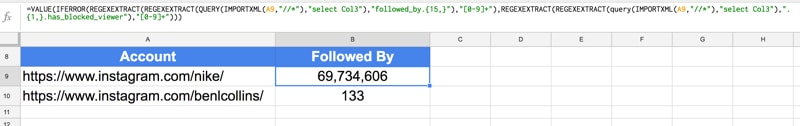See the Instagram Import Sheet.

##Import YouTube data

`https://www.youtube.com/benlcollins`

To get the number of subscribers to a YouTube channel, use this formula in cell B1:

`=VALUE(INDEX(REGEXEXTRACT(LOWER(INDEX(REGEXEXTRACT(INDEX(IMPORTXML(A1,"//div[@class='primary-header-actions']"),1,1),"(Unsubscribe)([0-9kmKM.]+)"),1,2)),"([0-9,.]+)([km]?)"),,1) * SWITCH(INDEX(REGEXEXTRACT(LOWER(INDEX(REGEXEXTRACT(INDEX(IMPORTXML(A1,"//div[@class='primary-header-actions']"),1,1),"(Unsubscribe)([0-9kmKM.]+)"),1,2)),"([0-9,.]+)([km]?)"),,2),"k",1000,"m",1000000,1))`

##Import Pinterest data

In cell A1, enter the following URL, again replacing benlcollins with the profile you’re interested in:

`https://www.pinterest.com/bencollins/`

Then in the adjacent cell, B1, enter the following formula:

`=IMPORTXML(A1,"//meta[@property='pinterestapp:followers']/@content")`

to get the following output (screenshot shows older version of the formula, latest one is above and in the template file):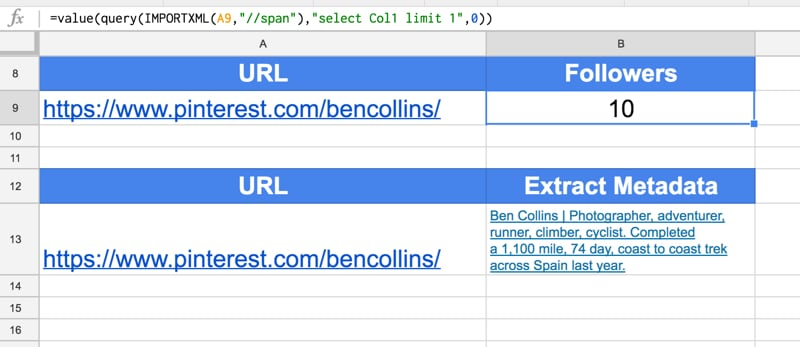Note, you can also get hold of the profile metadata with the import formulas, as follows:

`=IMPORTXML(A1,"//meta[@name='description']/@content")`

See the Pinterest Import Sheet.

##Import Alexa ranking data

Here there are two metrics I’m interested in – a site’s Global rank and a site’s US rank.

#### Global Rank

To get the Global rank for your site, enter your URL into cell A1 (replace benlcollins.com):

`http://www.alexa.com/siteinfo/benlcollins.com/`

and use the following helper formula in cell B1:

`=QUERY(ArrayFormula(QUERY(IMPORTDATA(A1),"select Col1") & QUERY(IMPORTDATA(A1),"select Col2")),"select * limit 1 offset " & MATCH(FALSE,ArrayFormula(ISNA(REGEXEXTRACT(QUERY(IMPORTDATA(A1),"select Col1") & QUERY(IMPORTDATA(A1),"select Col2"),"Global rank icon.{10,}"))),0)+1)`

and then extract the rank in cell C1:

`=VALUE(REGEXEXTRACT(B1,"[\d]{3,}"))`

#### US Rank

Assuming you have the Alexa URL in cell A1 again, then the US rank is extracted with this helper formula:

`=QUERY(ArrayFormula(QUERY(IMPORTDATA(A1),"select Col1") & QUERY(IMPORTDATA(A1),"select Col2")),"select * limit 1 offset " & MATCH(FALSE,ArrayFormula(ISNA(REGEXEXTRACT(QUERY(IMPORTDATA(A1),"select Col1") & QUERY(IMPORTDATA(A1),"select Col2"),"title='United States Flag'.alt.{50,}"))),0))`

and this formula to extract the actual rank value:

`=VALUE(REGEXEXTRACT(B1,"[\d]{3,}"))`

The following screenshot shows these formulas: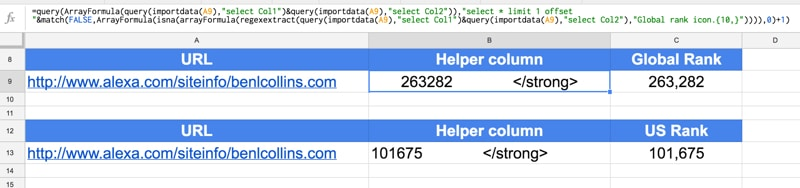See the Alexa Ranking Import Sheet.

^ Back to Contents

##Import Quora data

In this instance, I’ve imported the number followers Barack Obama has on Quora.

Quora is a little bit different because I need to use the URL and the profile name in my formula, so I’ve kept them in separate cells for that purpose. So in cell A1, add the generic Quora URL:

`https://www.quora.com/profile`/

And then in cell B1, add the profile name:

`Barack-Obama-44`

Then the formula in C1 to get the number of followers is:

`=VALUE(QUERY(IMPORTXML(A1&B1,"//a[@href='/profile/"&B1&"/followers']"),"select Col2"))`

The following screenshot shows this formula: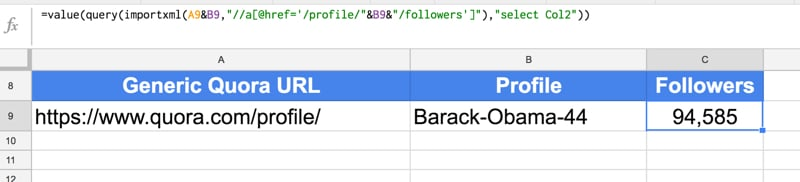See the Quora Import Sheet.

##Import Reddit data

Here, I’m using the funny subreddit as my example.

In A1:

`https://www.reddit.com/r/funny/`

To get the number of followers of this subreddit, use this formula in cell B1:

`=IMPORTXML(A1,"//span[@class='subscribers']/span[@class='number']")`

Bonus: To get the number of active viewers of this subreddit:

`=IMPORTXML(A1,"//p[@class='users-online']/span[@class='number']")`

The following screenshot shows these formulas: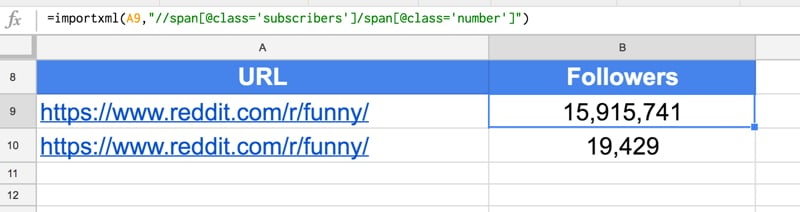See the Reddit Import Sheet.

##Import Spotify monthly listeners

Here’s a method for extracting the number of followers an artist has on the music streaming site Spotify.

First, find your favorite artist on Spotify: https://open.spotify.com/browse/featured

Copy the URL into cell A1 (it’ll look like this):

`https://open.spotify.com/artist/2ye2Wgw4gimLv2eAKyk1NB`

(This is Metallica, yeah 🤘)

Then put the following formula into cell A2 to extract the monthly listeners:

`=N(INDEX(IMPORTXML(A1,"//h3"),2))`

See the Spotify Import Sheet.

##Import Soundcloud data

`https://soundcloud.com/fleecemusic`

Here is the formula to extract page likes:

`=ArrayFormula(VALUE(REGEXEXTRACT(QUERY(SORT(IFERROR(REGEXEXTRACT( IMPORTXML(A1,"//script"),"followers_count..\d{1,}"),""),1,FALSE),"select * limit 1"),"\d{1,}")))`

Alternative formula:

Here is an alternative formula to extract the page metadata, which includes the likes:

`=IMPORTXML(A1,"//meta[@name='description']/@content")`

the formula to extract likes is:

`=VALUE(REGEXEXTRACT(REGEXEXTRACT(SUBSTITUTE( IMPORTXML(A1,"//meta[@name='description']/@content"),",",""),"\d{1,}.Followers"),"\d{1,}"))`

and to extract the “talking about” number:

`=VALUE(REGEXEXTRACT(REGEXEXTRACT(SUBSTITUTE( IMPORTXML(A1,"//meta[@name='description']/@content"),",",""),"\d{1,}.Tracks"),"\d{1,}"))`

The following screenshot shows these formulas: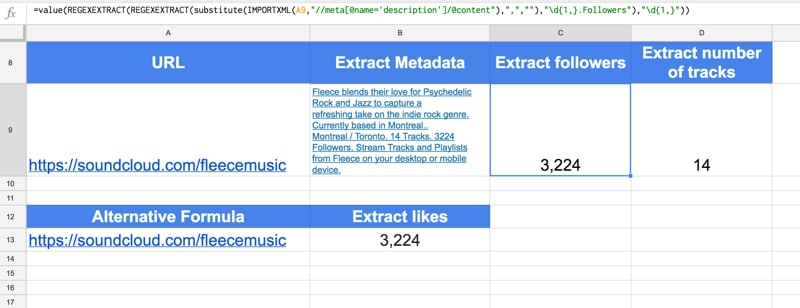See the Soundcloud Import Sheet.

##Import GTmetrix data

GTmetrix is a website that analyzes website performance.

You need to grab the correct URL before you can start scraping the data. So navigate to the GTmetrix site and enter the URL and hit analyze. You’ll end up with a URL like this:

`https://gtmetrix.com/reports/www.benlcollins.com/BcHv78bP`

Those last 8 characters (“BcHv78bP”) appear to be unique each time you run an analysis, so you’ll have to do this step manually.

Then in column B, I use this formula to extract the Page Speed Score and YSlow Score, into cells B1 and B2:

`=ArrayFormula(ABS(IMPORTXML(A1,"//span[@class='report-score-percent']")))`

and this formula in cell B3, to get the page details (Fully Loaded Time, Total Page Size and Requests) in cells B3, B4 and B5:

`=IMPORTXML(A1,"//span[@class='report-page-detail-value']")`

The following screenshot shows these formulas: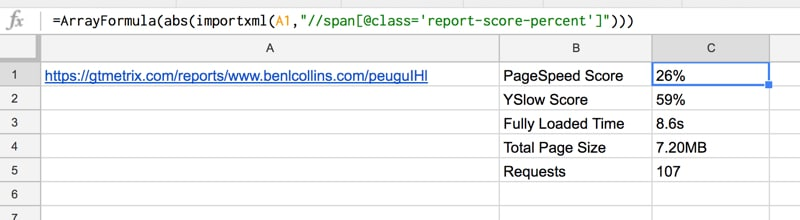See the GTmetrix Import Sheet.

##Import Bitly click data

Bitly is a service for shortening urls. They provide metrics for how many clicks you’ve had on each bitly link, e,g.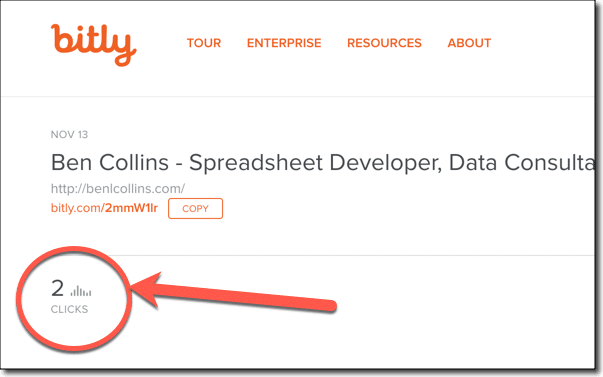Taking a standard Bitly link (e.g. http://bitly.com/2mmW1lr) and appending a “+” to it will take you to the dashboard page, with the metrics. Then we can use the import data function, a query function and a REGEX function to extract the click metrics.

User clicks are:

`=VALUE(REGEXEXTRACT(QUERY(IMPORTDATA(A9&"+"),"select Col1 limit 1"),"(?:user_clicks...)([0-9]+)"))`

and global clicks are:

`=VALUE(REGEXEXTRACT(QUERY(IMPORTDATA(A9&"+"),"select Col5"),"(?:global_clicks:.)([0-9]+)"))`

Clicks from the Bitly network are then simply the user clicks subtracted from the global clicks.

The following screenshot shows these formulas: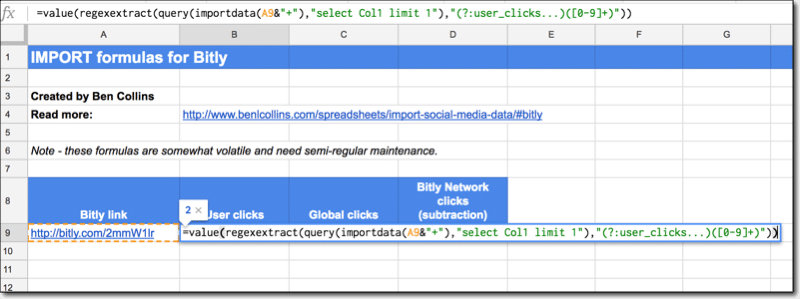See the Bitly Import Sheet.

##Import Linkedin data

This formula is no longer working for extracting Linkedin followers and I have not found an alternative.

In cell A1:

`https://www.linkedin.com/in/benlcollins/`

This formula used to work to get the number of Linkedin followers, but no longer:

`=QUERY(IMPORTXML(A1,"//div[@class='member-connections']"),"select Col1")`

and the output: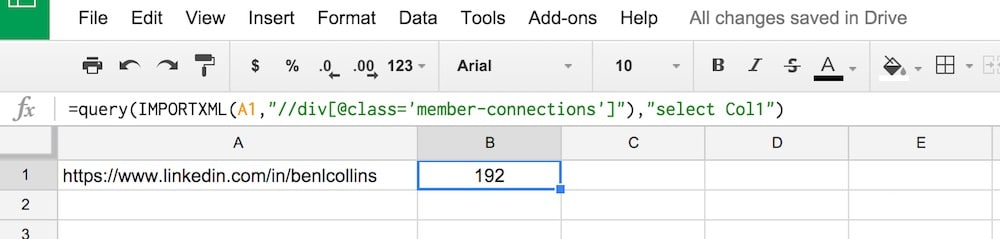There is no example sheet for Linkedin since the formula is no longer working.

##Sites that don’t work and why not

I’ve tried the following sites but the IMPORT formulas are unable to extract the social media statistics:

• Similar Web
• Twitch
• Mobcrush
• Crunchbase
• Angel.co
• Majestic SEO

These are all modern sites built using front-end, client-side Javascript frameworks, so the IMPORT formulas can’t extract any data because the page is built dynamically in browser as it’s loaded up. The IMPORT formulas work fine on sites built in the traditional fashion, with lots of well formed HTML tags, where the social media statistics are embedded into the site markup that is passed from the server.

Compare this screenshot of the source code for Mobcrush, built using Angular JS it looks like (click to enlarge):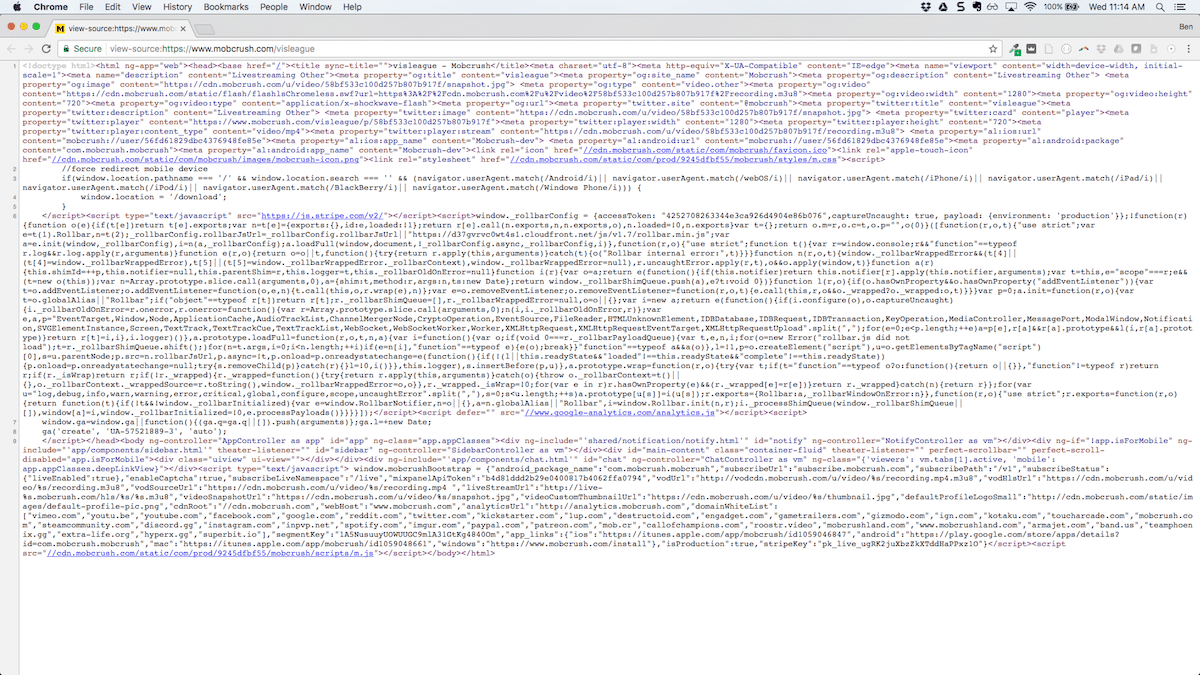versus what the source code looks for this page on my website (click to enlarge):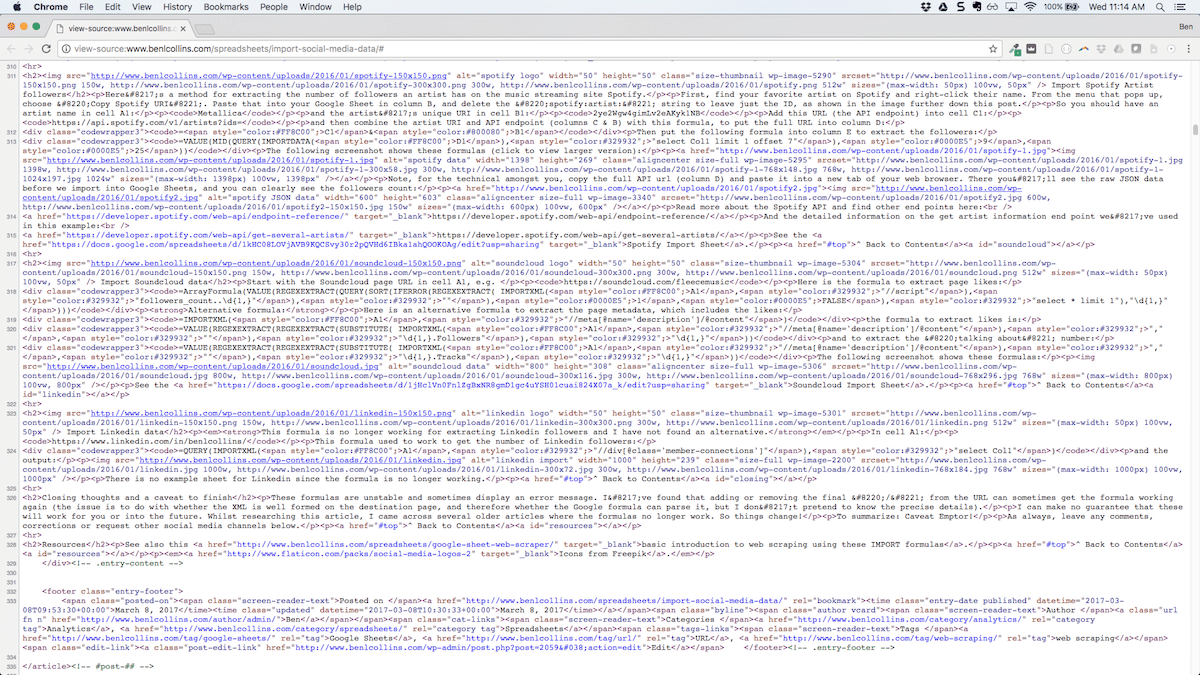You can see the code for my site has lots of tags which the IMPORT formulas can parse, whereas the other site’s code does not.

If anyone knows of any clever way to get around this, do share!

Otherwise, you’re next option is to venture down the API route. Yes, this involves coding, but it’s not as hard as you think.

I’ll be posting some API focussed articles soon. In the meantime, check out my post on how to get started with APIs, or for a peak at what’s coming, take a look at my Apps Script + API repo on GitHub.

Also, even when these formulas are working, they can be temperamental. If you work with them a lot, sooner or later you’ll find yourself hitting this loading issue all the time, where the formulas stop displaying any results: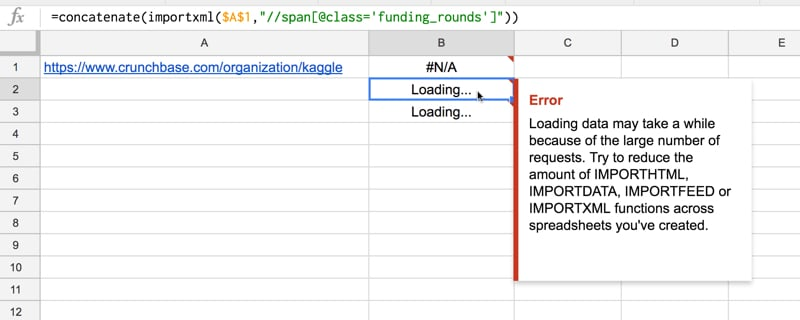##Closing thoughts

These formulas are unstable and will sometimes display an error message.

I’ve found that adding or removing the final “/” from the URL can sometimes get the formula working again (the issue is to do with caching).

I can make no guarantee that these will work for you or into the future. Whilst researching this article, I came across several older articles where many of the formulas no longer work. So things change!

To summarize: Caveat Emptor!

##Resources

As always, leave any comments, corrections or request other social media statistics below.

Icons from Freepik.